# Text Classification

Text classification is an extremely popular task. You enjoy working text classifiers in your mail agent: it classifies letters and filters spam. Other applications include document classification, review classification, etc.

Text classifiers are often used not as an individual task, but as part of bigger pipelines. For example, a voice assistant classifies your utterance to understand what you want (e.g., set the alarm, order a taxi or just chat) and passes your message to different models depending on the classifier's decision. Another example is a web search engine: it can use classifiers to identify the query language, to predict the type of your query (e.g., informational, navigational, transactional), to understand whether you what to see pictures or video in addition to documents, etc.

Since most of the classification datasets assume that only one label is correct (you will see this right now!), in the lecture we deal with this type of classification, i.e. the single-label classification. We mention multi-label classification in a separate section (Multi-Label Classification).

## Datasets for Classification

Datasets for text classification are very different in terms of size (both dataset size and examples' size), what is classified, and the number of labels. Look at the statistics below.

Dataset Type Number
of labels
Size
(train/test)
Avg. length
(tokens)
SST sentiment 5 or 2 8.5k / 1.1k 19
IMDb Review sentiment 2 25k / 25k 271
Yelp Review sentiment 5 or 2 650k / 50k 179
Amazon Review sentiment 5 or 2 3m / 650k 79
TREC question 6 5.5k / 0.5k 10
Yahoo! Answers question 10 1.4m / 60k 131
AG’s News topic 4 120k / 7.6k 44
Sogou News topic 6 54k / 6k 737
DBPedia topic 14 560k / 70k 67

The most popular datasets are for sentiment classification. They consist of reviews of movies, places or restaurants, and products. There are also datasets for question type classification and topic classification.

To better understand typical classification tasks, below you can look at the examples from different datasets.How to: pick a dataset and look at the examples to get a feeling of the task. Or you can come back to this later!

Pick a dataset

Dataset Description (click!)

SST is a sentiment classification dataset which consists of movie reviews (from Rotten Tomatoes html files). The dataset consists of parse trees of the sentences, and not only entire sentences, but also smaller phrases have a sentiment label.

There are five labels: 1 (very negative), 2 (negative), 3 (neutral), 4 (positive), and 5 (very positive) (alternatively, labels can be 0-4). Depending on the used labels, you can get either binary SST-2 dataset (if you only consider positivity and negativity) or fine-grained sentiment SST-5 (when using all labels).

Note that the dataset size mentioned above (8.5k/2.2k/1.1k for train/dev/test) is in the number of sentences. However, it also has 215,154 phrases that compose each sentence in the dataset.

For more details, see the original paper.

Look how sentiment of a sentence is composed from its parts.

Dataset Description (click!)

IMDb is a large dataset of informal movie reviews from the Internet Movie Database. The collection allows no more than 30 reviews per movie. The dataset contains an even number of positive and negative reviews, so randomly guessing yields 50% accuracy. The reviews are highly polarized: they are only negative (with the highest score 4 out of 10) or positive (with the lowest score 7 out of 10).

For more details, see the original paper.

Dataset Description (click!)

The Yelp reviews dataset is obtained from the Yelp Dataset Challenge in 2015. Depending on the number of labels, you can get either Yelp Full (all 5 labels) or Yelp Polarity (positive and negative classes) dataset. Full has 130,000 training samples and 10,000 testing samples in each star, and the Polarity dataset has 280,000 training samples and 19,000 test samples in each polarity.

For more details, see the Kaggle Challenge page.

Dataset Description (click!)

The Amazon reviews dataset consists of reviews from amazon which include product and user information, ratings, and a plaintext review. The dataset is obtained from the Stanford Network Analysis Project (SNAP). Depending on the number of labels, you can get either Amazon Full (all 5 labels) or Amazon Polarity (positive and negative classes) dataset. Full has 600,000 training samples and 130,000 testing samples in each star, and the Polarity dataset has 1800,000 training samples and 200,000 test samples in each polarity. The fields used are review title and review content.

Dataset Description (click!)

TREC is a dataset for classification of free factual questions. It defines a two-layered taxonomy, which represents a natural semantic classification for typical answers in the TREC task. The hierarchy contains 6 coarse classes (ABBREVIATION, ENTITY, DESCRIPTION, HUMAN, LOCATION and NUMERIC VALUE) and 50 fine classes.

For more details, see the original paper.

Dataset Description (click!)

The dataset is gathered from Yahoo! Answers Comprehensive Questions and Answers version 1.0 dataset. In contains the 10 largest main categories: "Society & Culture", "Science & Mathematics", "Health, "Education & Reference", "Computers & Internet", "Sports", "Business & Finance", "Entertainment & Music", "Family & Relationships", "Politics & Government". Each class contains 140,000 training samples and 5,000 testing samples. The data consists of question title and content, as well as the best answer.

Dataset Description (click!)

The AG’s corpus was obtained from news articles on the web. From these articles, only the AG’s corpus contains only the title and description fields from the the 4 largest classes.

The dataset was introduced in this paper.

Dataset Description (click!)

The Sogou News corpus was obtained from the combination of the SogouCA and SogouCS news corpora. The dataset consists of news articles (title and content fields) labeled with 5 categories: “sports”, “finance”, “entertainment”, “automobile” and “technology”.

The original dataset is in Chinese, but you can produce Pinyin – a phonetic romanization of Chinese. You can do it using pypinyin package combined with jieba Chinese segmentation system (this is what the paper introducing the dataset did, and this is what I show you in the examples). The models for English can then be applied to this dataset without change.

The dataset was introduced in this paper, the dataset in Pinyin can be downloaded here.

Lena: Here I picked very small texts - usually, the samples are much longer.

Dataset Description (click!)

DBpedia is a crow-sourced community effort to extract structured information from Wikipedia. The DBpedia ontology classification dataset is constructed by picking 14 non-overlapping classes from DBpedia 2014. From each of the 14 ontology classes, the dataset contains 40,000 randomly chosen training samples and 5,000 testing samples. Therefore, the total size of the training dataset is 560,000, the testing dataset - 70,000.

The dataset was introduced in this paper.# General View

Here we provide a general view on classification and introduce the notation. This section applies to both classical and neural approaches.

We assume that we have a collection of documents with ground-truth labels. The input of a classifier is a document $$x=(x_1, \dots, x_n)$$ with tokens $$(x_1, \dots, x_n)$$, the output is a label $$y\in 1\dots k$$. Usually, a classifier estimates probability distribution over classes, and we want the probability of the correct class to be the highest.

## Get Feature Representation and Classify

Text classifiers have the following structure: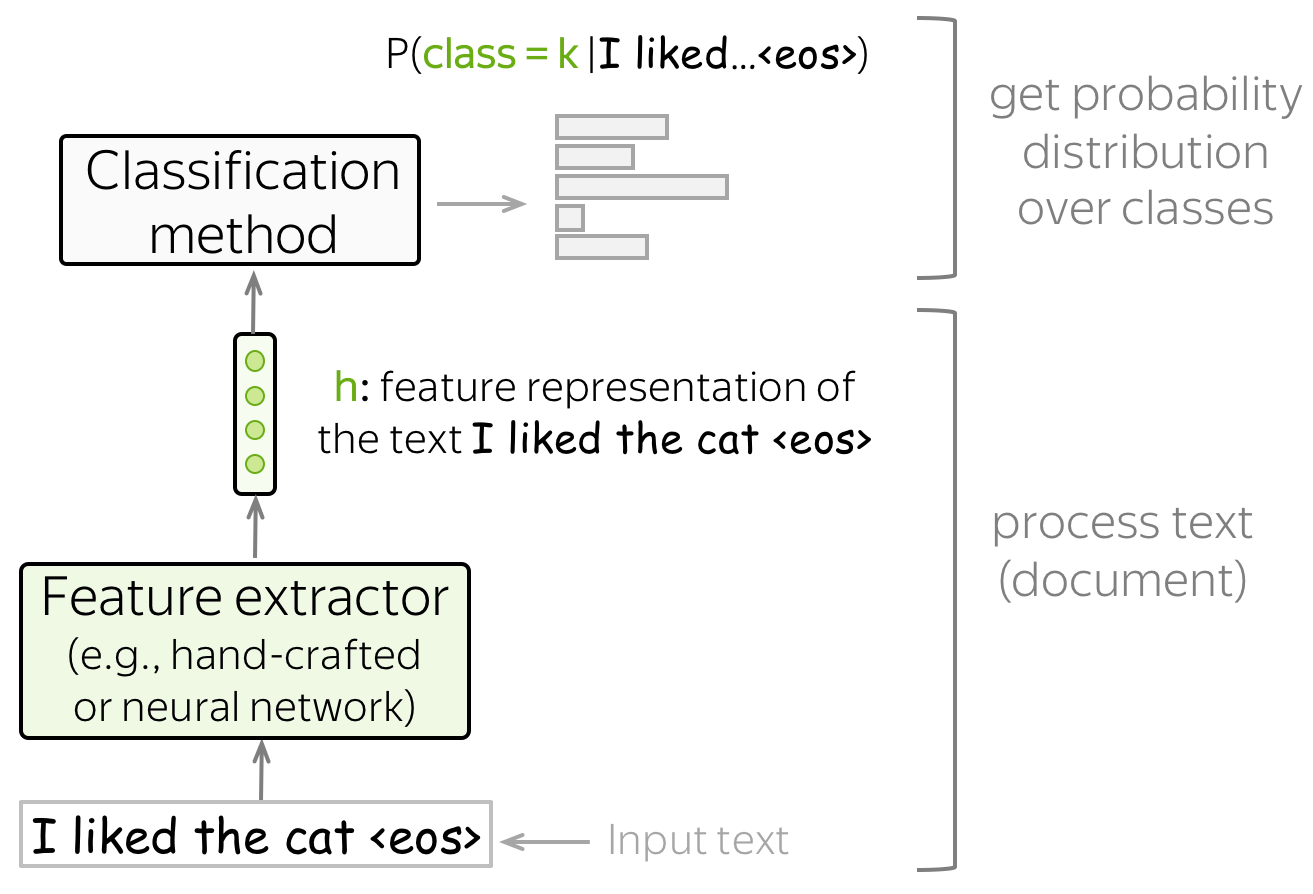• feature extractor
A feature extractor can be either manually defined (as in classical approaches) or learned (e.g., with neural networks).
• classifier
A classifier has to assign class probabilities given feature representation of a text. The most common way to do this is using logistic regression, but other variants are also possible (e.g., Naive Bayes classifier or SVM).

In this lecture, we'll mostly be looking at different ways to build feature representation of a text and to use this representation to get class probabilities.

## Generative and Discriminative Models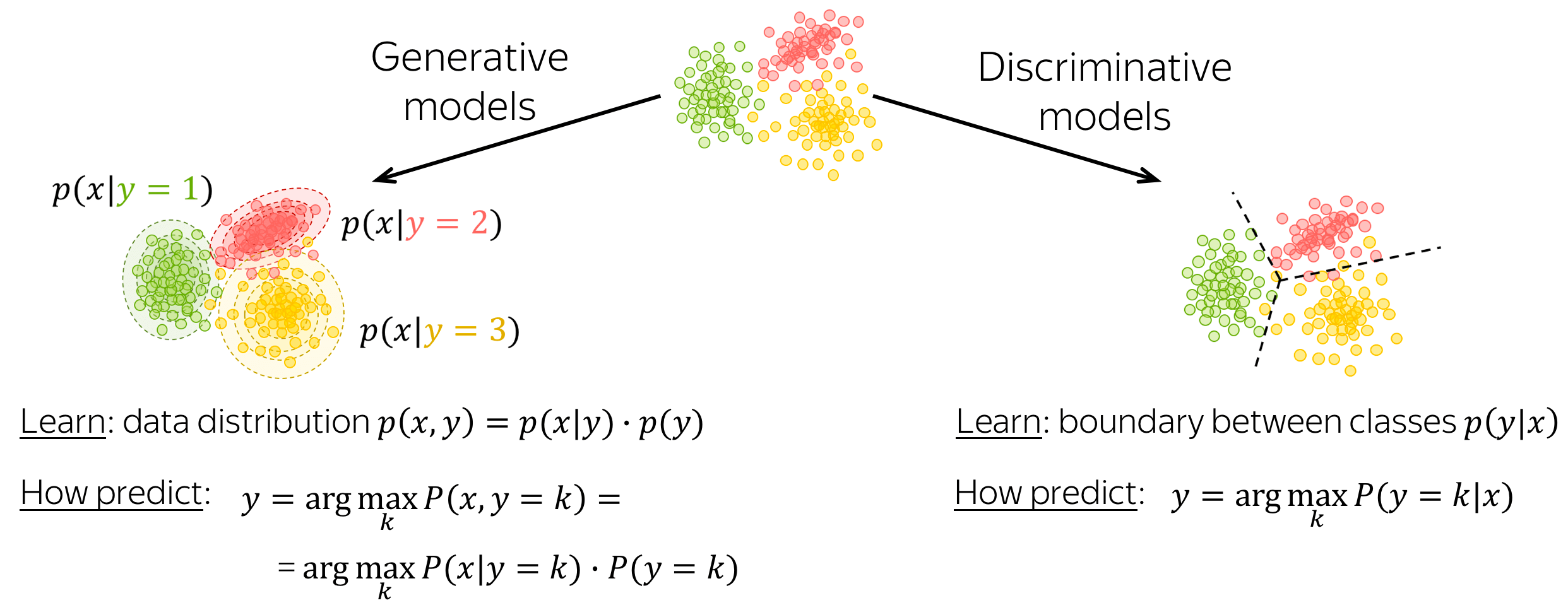A classification model can be either generative or discriminative.

• generative models
Generative models learn joint probability distribution of data $$p(x, y) = p(x|y)\cdot p(y)$$. To make a prediction given an input $$x$$, these models pick a class with the highest joint probability: $$y = \arg \max\limits_{k}p(x|y=k)\cdot p(y=k)$$.
• discriminative models
Discriminative models are interested only in the conditional probability $$p(y|x)$$, i.e. they learn only the border between classes. To make a prediction given an input $$x$$, these models pick a class with the highest conditional probability: $$y = \arg \max\limits_{k}p(y=k|x)$$.

In this lecture, we will meet both generative and discriminative models.

# Classical Methods for Text Classification

In this part, we consider classical approaches for text classification. They were developed long before neural networks became popular, and for small datasets can still perform comparably to neural models.

Lena: Later in the course, we will learn about transfer learning which can make neural approaches better even for very small datasets. But let's take this one step at a time: for now, classical approaches are a good baseline for your models.

## Naive Bayes Classifier

A high-level idea of the Naive Bayes approach is given below: we rewrite the conditional class probability $$P(y=k|x)$$ using Bayes's rule and get $$P(x|y=k)\cdot P(y=k)$$.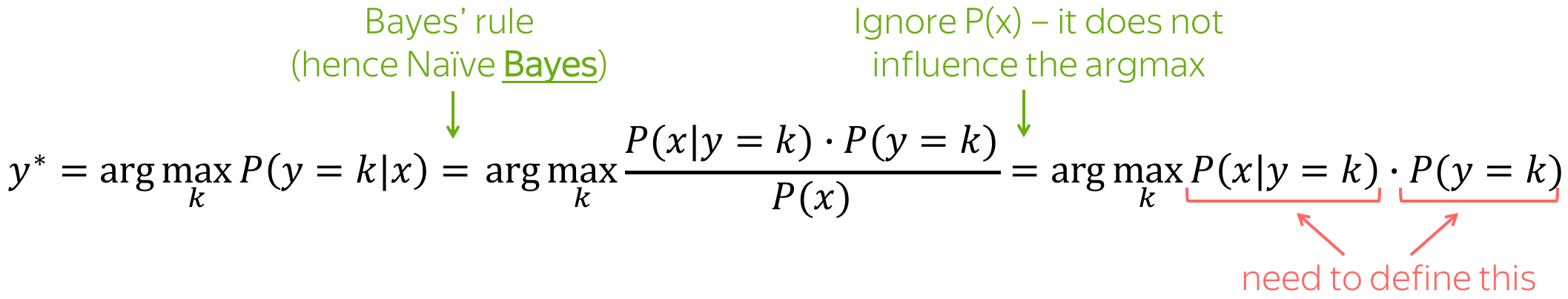### This is a generative model!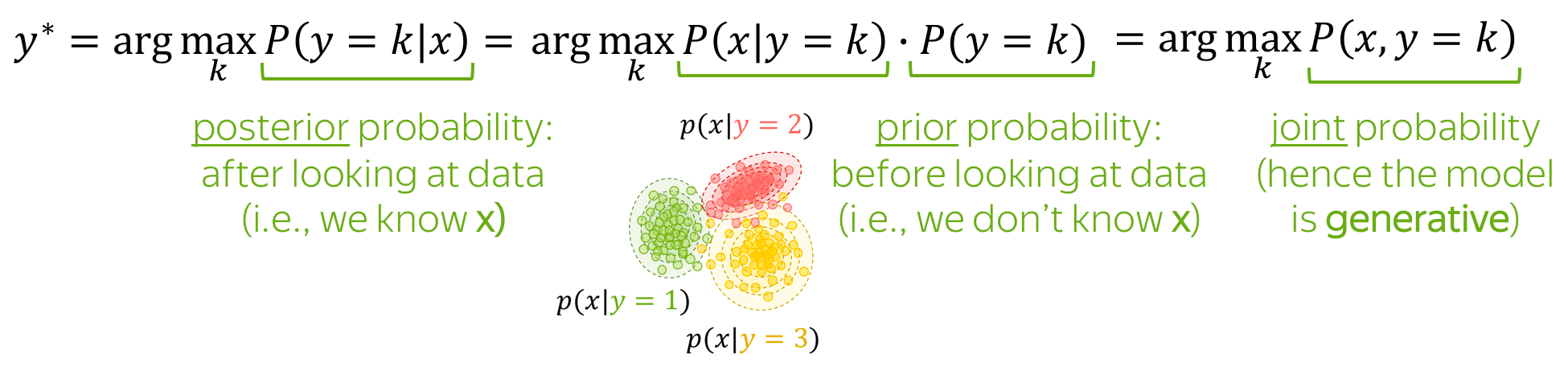Naive Bayes is a generative model: it models the joint probability of data.

Note also the terminology:

• prior probability $$P(y=k)$$: class probability before looking at data (i.e., before knowing $$x$$);
• posterior probability $$P(y=k|x)$$: class probability after looking at data (i.e., after knowing the specific $$x$$);
• joint probability $$P(x, y)$$: the joint probability of data (i.e., both examples $$x$$ and labels $$y$$);
• maximum a posteriori (MAP) estimate: we pick the class with the highest posterior probability.

### P(y=k): count labels

$$P(y=k)$$ is very easy to get: we can just evaluate the proportion of documents with the label $$k$$ (this is the maximum likelihood estimate, MLE). Namely, $P(y=k)=\frac{N(y=k)}{\sum\limits_{i}N(y=i)},$ where $$N(y=k)$$ is the number of examples (documents) with the label $$k$$.

### P(x|y=k): use the "naive" assumptions, then count

Here we assume that document $$x$$ is represented as a set of features, e.g., a set of its words $$(x_1, \dots, x_n)$$: $P(x| y=k)=P(x_1, \dots, x_n|y=k).$

The Naive Bayes assumptions are

• Bag of Words assumption: word order does not matter,
• Conditional Independence assumption: features (words) are independent given the class.

Intuitively, we assume that the probability of each word to appear in a document with class $$k$$ does not depend on context (neither word order nor other words at all). For example, we can say that awesome, brilliant, great are more likely to appear in documents with a positive sentiment and awful, boring, bad are more likely in negative documents, but we know nothing about how these (or other) words influence each other.

With these "naive" assumptions we get: $P(x| y=k)=P(x_1, \dots, x_n|y=k)=\prod\limits_{t=1}^nP(x_t|y=k).$ The probabilities $$P(x_i|y=k)$$ are estimated as the proportion of times the word $$x_i$$ appeared in documents of class $$k$$ among all tokens in these documents: $P(x_i|y=k)=\frac{N(x_i, y=k)}{\sum\limits_{t=1}^{|V|}N(x_t, y=k)},$ where $$N(x_i, y=k)$$ is the number of times the token $$x_i$$ appeared in documents with the label $$k$$, $$V$$ is the vocabulary (more generally, a set of all possible features).

### What if $$N(x_i, y=k)=0$$? Need to avoid this!

What if $$N(x_i, y=k)=0$$, i.e. in training we haven't seen the token $$x_i$$ in the documents with class $$k$$? This will null out the probability of the whole document, and this is not what we want! For example, if we haven't seen some rare words (e.g., pterodactyl or abracadabra) in training positive examples, it does not mean that a positive document can never contain these words.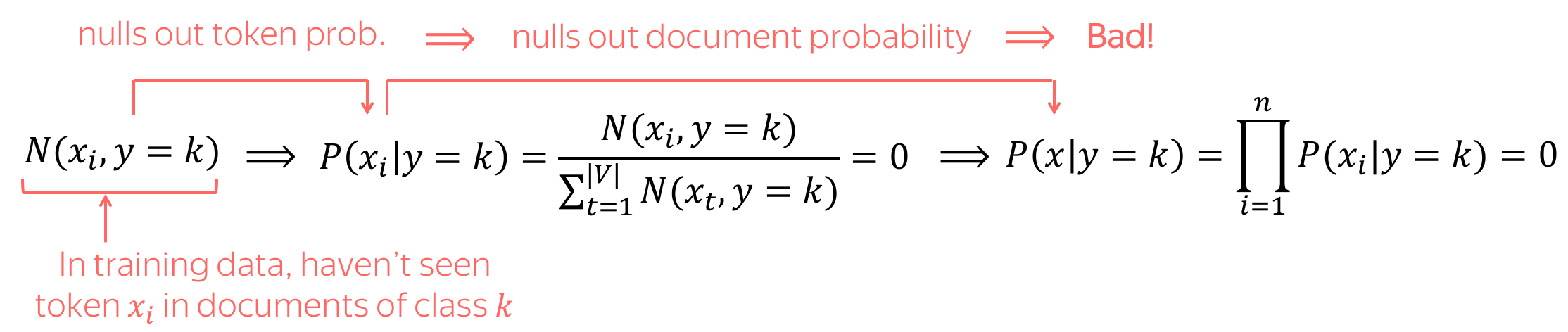To avoid this, we'll use a simple trick: we add to counts of all words a small $$\delta$$: $P(x_i|y=k)=\frac{\color{red}{\delta} +\color{black} N(x_i, y=k) }{\sum\limits_{t=1}^{|V|}(\color{red}{\delta} +\color{black}N(x_t, y=k))} = \frac{\color{red}{\delta} +\color{black} N(x_i, y=k) }{\color{red}{\delta\cdot |V|}\color{black} + \sum\limits_{t=1}^{|V|}\color{black}N(x_t, y=k)} ,$ where $$\delta$$ can be chosen using cross-validation.

Note: this is Laplace smoothing (aka Add-1 smoothing if $$\delta=1$$). We'll learn more about smoothings in the next lecture when talking about Language Modeling.

### Making a Prediction

As we already mentioned, Naive Bayes (and, more broadly, generative models) make a prediction based on the joint probability of data and class: $y^{\ast} = \arg \max\limits_{k}P(x, y=k) = \arg \max\limits_{k} P(y=k)\cdot P(x|y=k).$

Intuitively, Naive Bayes expects that some words serve as class indicators. For example, for sentiment classification tokens awesome, brilliant, great will have higher probability given positive class then negative. Similarly, tokens awful, boring, bad will have higher probability given negative class then positive.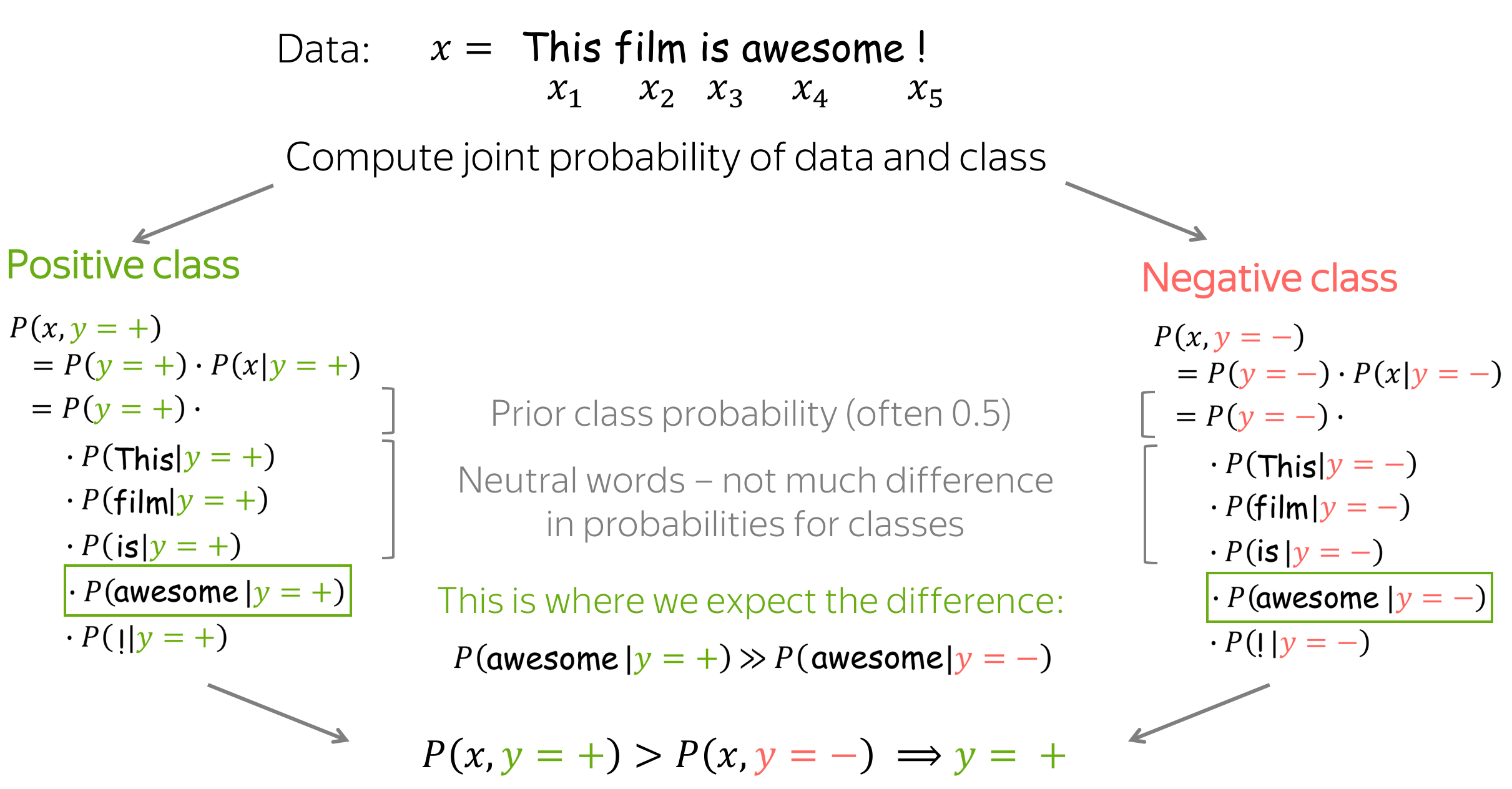### Practical Note: Sum of Log-Probabilities Instead of Product of Probabilities

The main expression Naive Bayes uses for classification is a product lot of probabilities: $P(x, y=k)=P(y=k)\cdot P(x_1, \dots, x_n|y)=P(y=k)\cdot \prod\limits_{t=1}^nP(x_t|y=k).$ A product of many probabilities may be very unstable numerically. Therefore, usually instead of $$P(x, y)$$ we consider $$\log P(x, y)$$: $\log P(x, y=k)=\log P(y=k) + \sum\limits_{t=1}^n\log P(x_t|y=k).$ Since we care only about argmax, we can consider $$\log P(x, y)$$ instead of $$P(x, y)$$.

Important! Note that in practice, we will usually deal with log-probabilities and not probabilities.

### View in the General Framework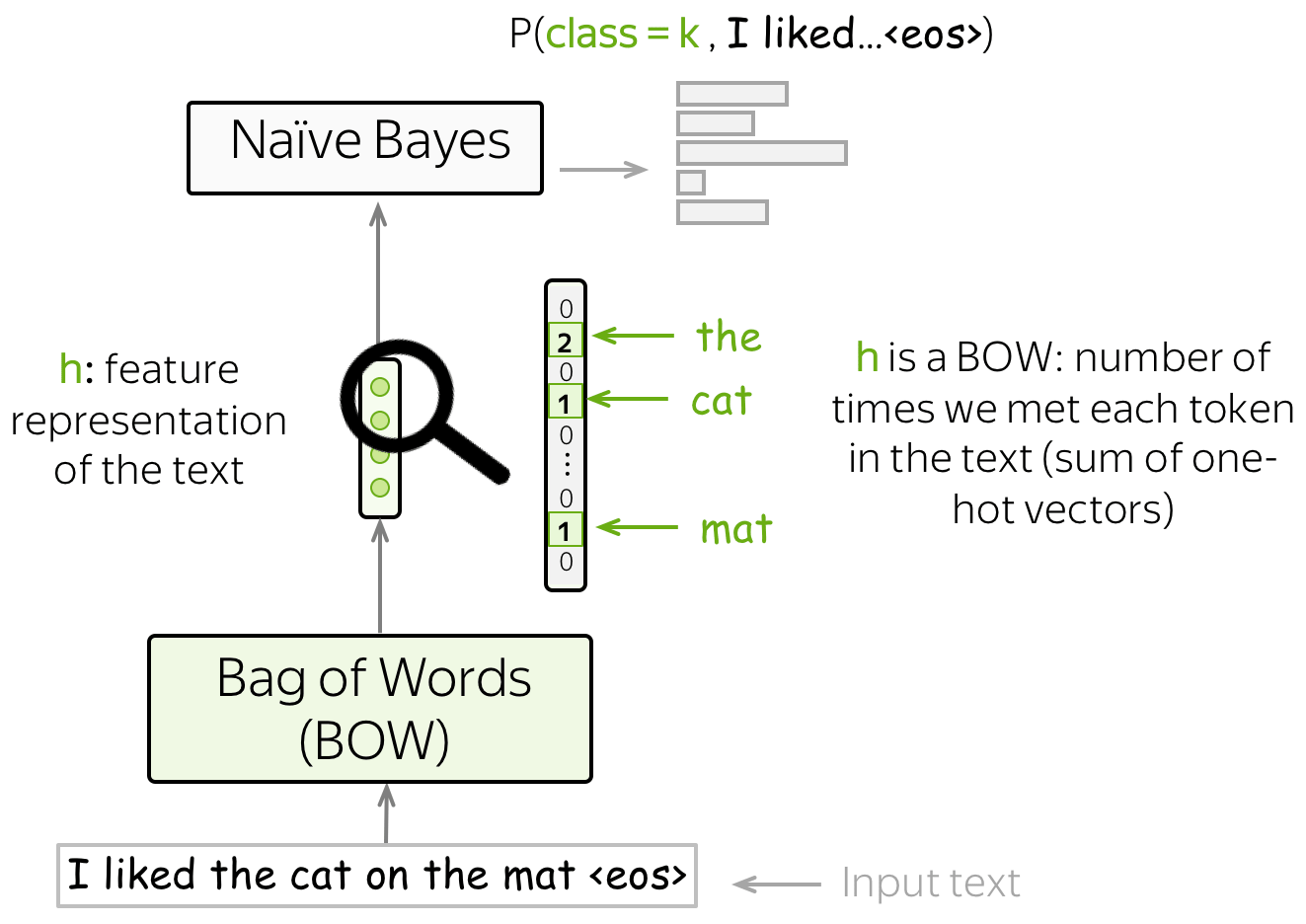Remember our general view on the classification task? We obtain feature representation of the input text using some method, then use this feature representation for classification.

In Naive Bayes, our features are words, and the feature representation is the Bag-of-Words (BOW) representation - a sum of one-hot representations of words. Indeed, to evaluate $$P(x, y)$$ we only need to count the number of times each token appeared in the text.

### Feature Design

In the standard setting, we used words as features. However, you can use other types of features: URL, user id, etc.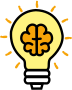Even if your data is a plain text (without fancy things such as URL, user id, etc), you can still design features in different ways. Learn how to improve Naive Bayes in this exercise in the Research Thinking section.

## Maximum Entropy Classifier (aka Logistic Regression)

Differently from Naive Bayes, MaxEnt classifier is a discriminative model, i.e., we are interested in $$P(y=k|x)$$ and not in the joint distribution $$p(x, y)$$. Also, we will learn how to use features: this is in contrast to Naive Bayes, where we defined how to use the features ourselves.

Here we also have to define features manually, but we have more freedom: features do not have to be categorical (in Naive Bayes, they had to!). We can use the BOW representation or come up with something more interesting.

The general classification pipeline here is as follows:

• get $$\color{#7aab00}{h}\color{black}=(\color{#7aab00}{f_1}\color{black}, \color{#7aab00}{f_2}\color{black}, \dots, \color{#7aab00}{f_n}\color{black}{)}$$ - feature representation of the input text;
• take $$w^{(i)}=(w_1^{(i)}, \dots, w_n^{(i)})$$ - vectors with feature weights for each of the classes;
• for each class, weigh features, i.e. take the dot product of feature representation $$\color{#7aab00}{h}$$ with feature weights $$w^{(k)}$$: $w^{(k)}\color{#7aab00}{h}\color{black} = w_1^{(k)}\cdot\color{#7aab00}{f_1}\color{black}+\dots+ w_n^{(k)}\cdot\color{#7aab00}{f_n}\color{black}{, \ \ \ \ \ k=1, \dots, K.}$ To get a bias term in the sum above, we define one of the features being 1 (e.g., $$\color{#7aab00}{f_0}=1$$). Then $w^{(k)}\color{#7aab00}{h}\color{black} = \color{red}{w_0^{(k)}}\color{black} + w_1^{(k)}\cdot\color{#7aab00}{f_1}\color{black}+\dots+ w_n^{(k)}\cdot\color{#7aab00}{f_{n}}\color{black}{, \ \ \ \ \ k=1, \dots, K.}$
• get class probabilities using softmax: $P(class=k|\color{#7aab00}{h}\color{black})= \frac{\exp(w^{(k)}\color{#7aab00}{h}\color{black})}{\sum\limits_{i=1}^K \exp(w^{(i)}\color{#7aab00}{h}\color{black})}.$ Softmax normalizes the $$K$$ values we got at the previous step to a probability distribution over output classes.

Look at the illustration below (classes are shown in different colors).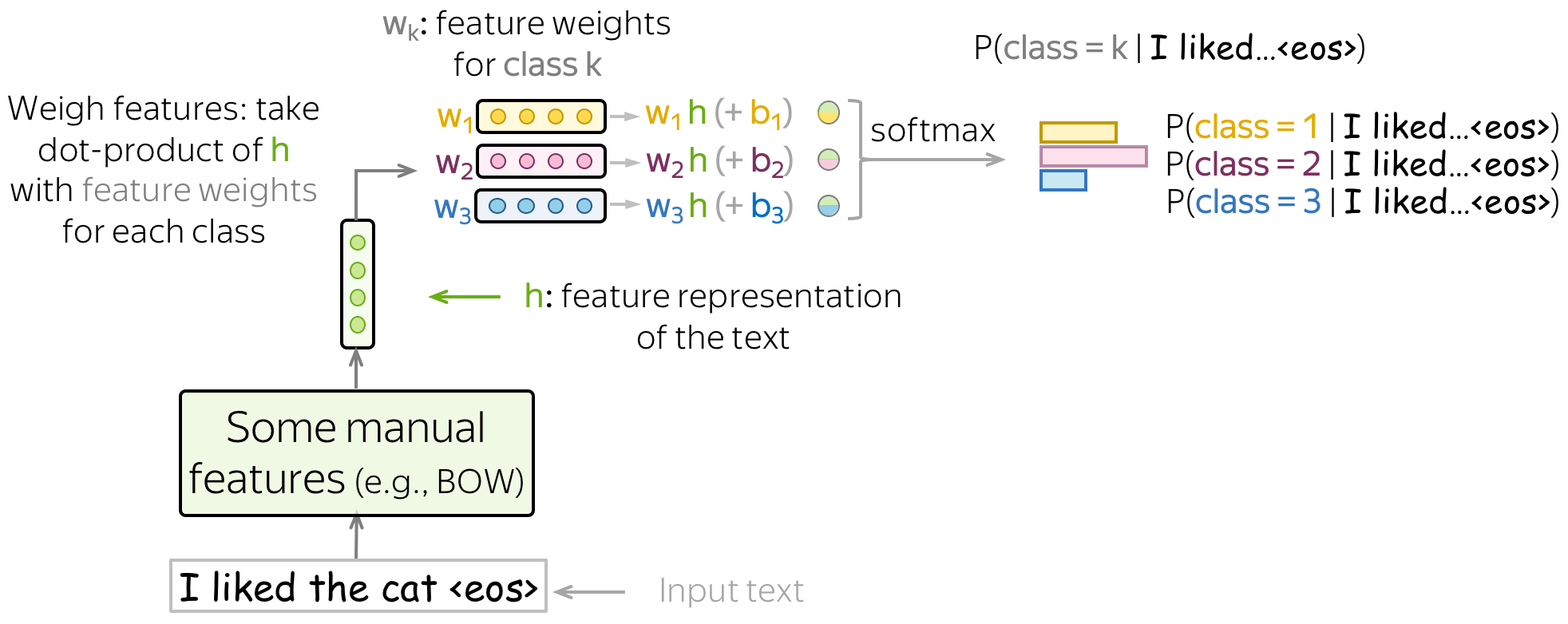### Training: Maximum Likelihood Estimate

Given training examples $$x^1, \dots, x^N$$ with labels $$y^1, \dots, y^N$$, $$y^i\in\{1, \dots, K\}$$, we pick those weights $$w^{(k)}, k=1..K$$ which maximize the probability of the training data: $w^{\ast}=\arg \max\limits_{w}\sum\limits_{i=1}^N\log P(y=y^i|x^i).$ In other words, we choose parameters such that the data is more likely to appear. Therefore, this is called the Maximum Likelihood Estimate (MLE) of the parameters.

To find the parameters maximizing the data log-likelihood, we use gradient ascent: gradually improve weights during multiple iterations over the data. At each step, we maximize the probability a model assigns to the correct class.

### Equvalence to minimizing cross-entropy

Note that maximizing data log-likelihood is equivalent to minimizing cross entropy between the target probability distribution $$p^{\ast} = (0, \dots, 0, 1, 0, \dots)$$ (1 for the target label, 0 for the rest) and the predicted by the model distribution $$p=(p_1, \dots, p_K), p_i=p(i|x)$$: $Loss(p^{\ast}, p^{})= - p^{\ast} \log(p) = -\sum\limits_{i=1}^{K}p_i^{\ast} \log(p_i).$ Since only one of $$p_i^{\ast}$$ is non-zero (1 for the target label $$k$$, 0 for the rest), we will get $$Loss(p^{\ast}, p) = -\log(p_{k})=-\log(p(k| x)).$$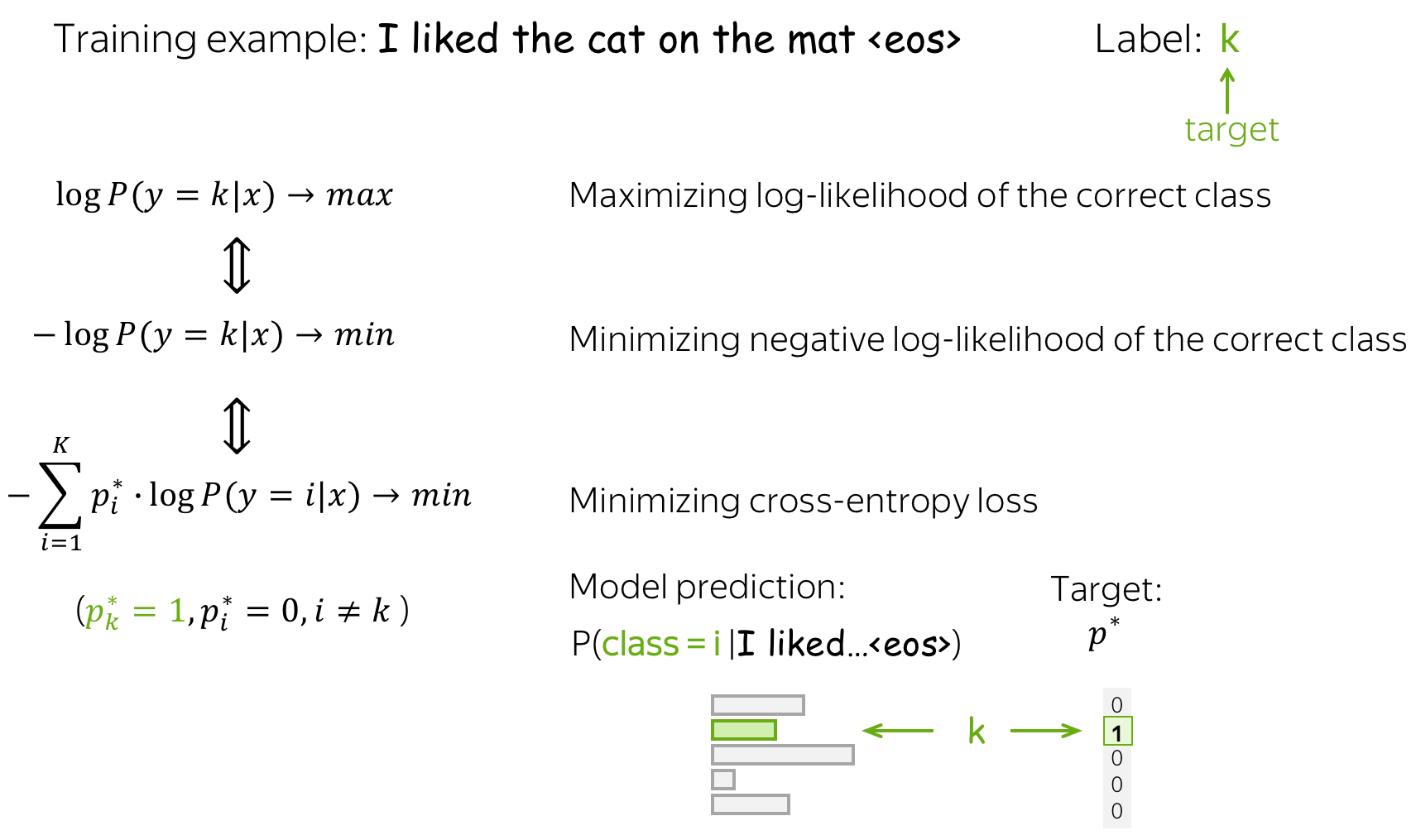This equivalence is very important for you to understand: when talking about neural approaches, people usually say that they minimize the cross-entropy loss. Do not forget that this is the same as maximizing the data log-likelihood.

### Naive Bayes vs Logistic Regression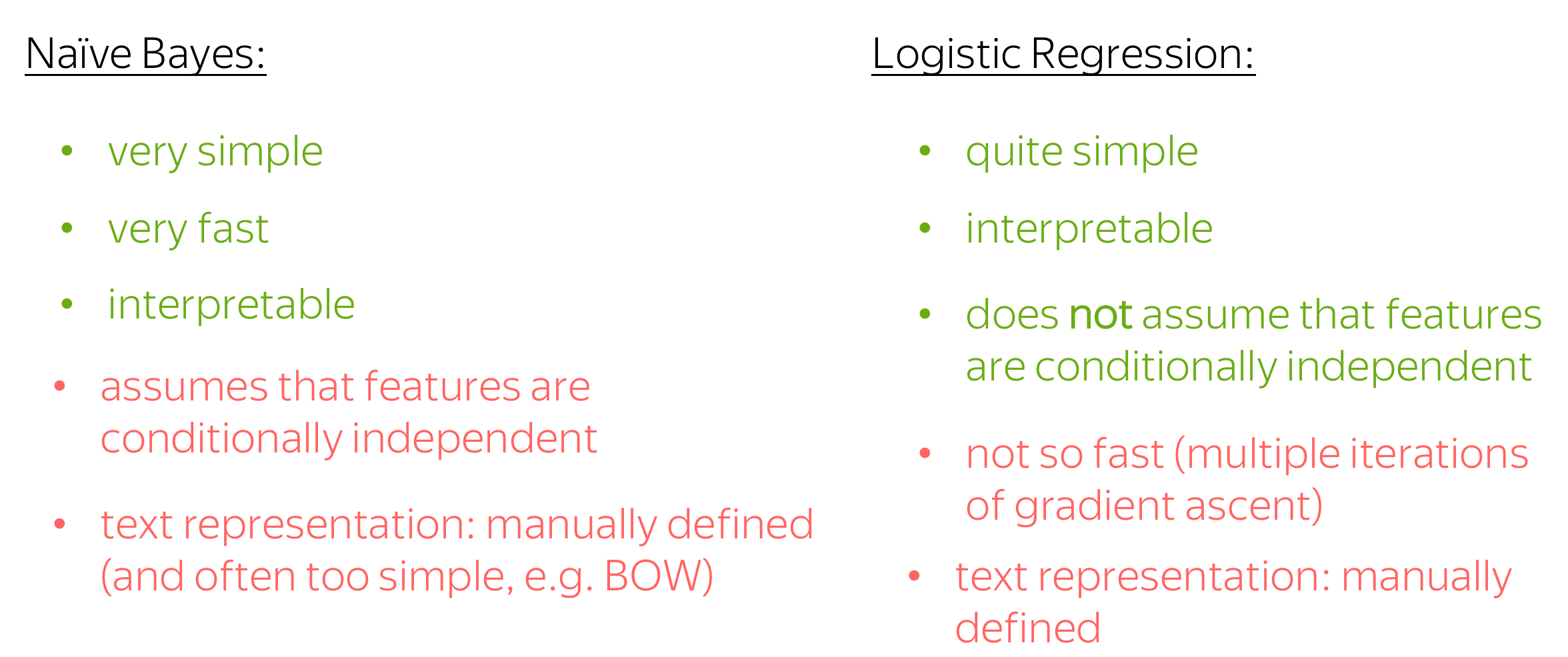Let's finalize this part by discussing the advantages and drawbacks of logistic regression and Naive Bayes.

• simplicity
Both methods are simple; Naive Bayes is the simplest one.
• interpretability
Both methods are interpretable: you can look at the features which influenced the predictions most (in Naive Bayes - usually words, in logistic regression - whatever you defined).
• training speed
Naive Bayes is very fast to train - it requires only one pass through the training data to evaluate the counts. For logistic regression, this is not the case: you have to go over the data many times until the gradient ascent converges.
• independence assumptions
Naive Bayes is too "naive" - it assumed that features (words) are conditionally independent given class. Logistic regression does not make this assumption - we can hope it is better.
• text representation: manual
Both methods use manually defined feature representation (in Naive Bayes, BOW is the standard choice, but you still choose this yourself). While manually defined features are good for interpretability, they may be no so good for performance - you are likely to miss something which can be useful for the task.

## SVM for Text Classification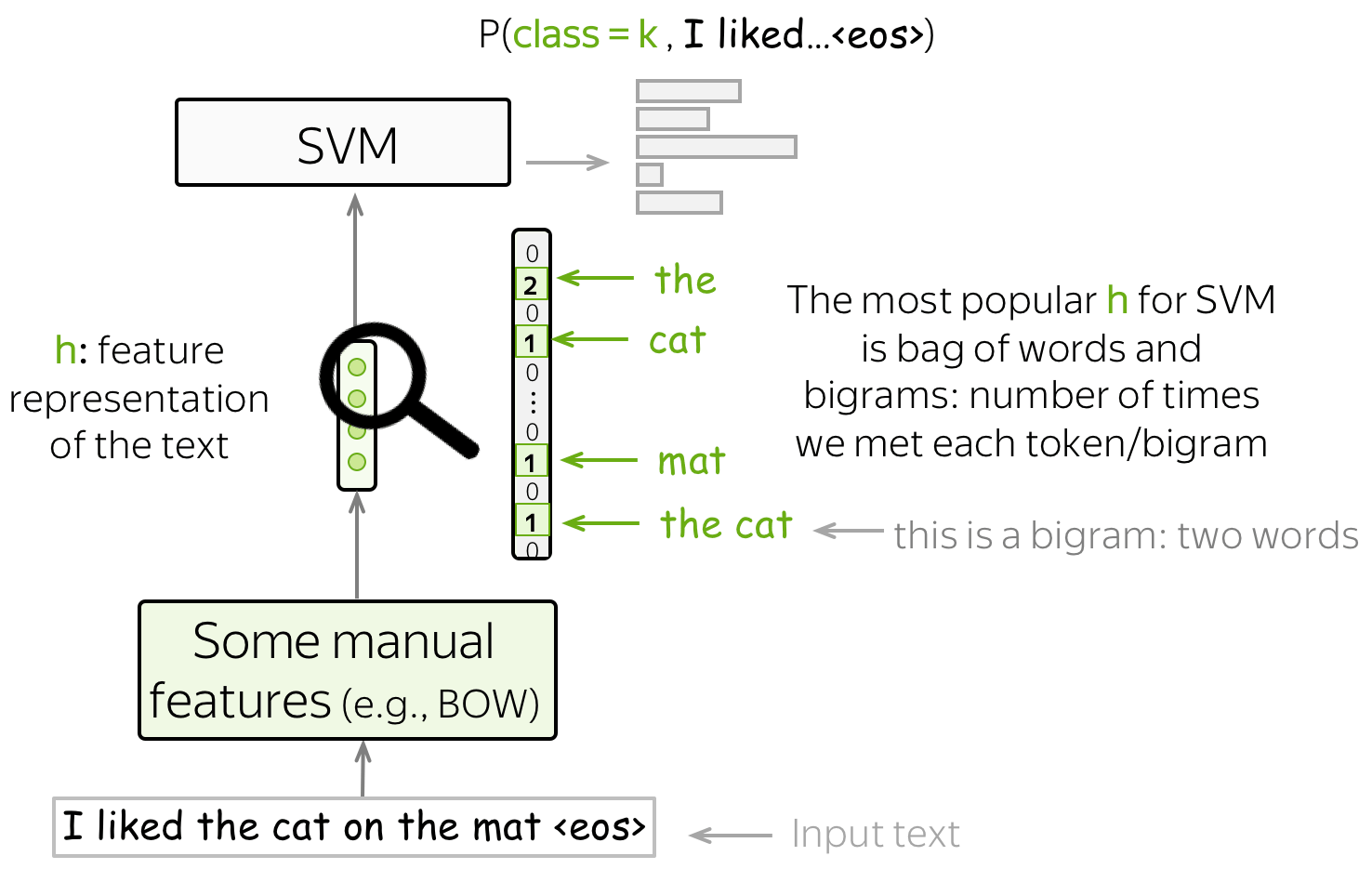One more method for text classification based on manually designed features is SVM. The most basic (and popular) features for SVMs are bag-of-words and bag-of-ngrams (ngram is a tuple of n words). With these simple features, SVMs with linear kernel perform better than Naive Bayes (see, for example, the paper Question Classification using Support Vector Machines).

# Text Classification with Neural Networks

Instead of manually defined features, let a neural network to learn useful features.

The main idea of neural-network-based classification is that feature representation of the input text can be obtained using a neural network. In this setting, we feed the embeddings of the input tokens to a neural network, and this neural network gives us a vector representation of the input text. After that, this vector is used for classification.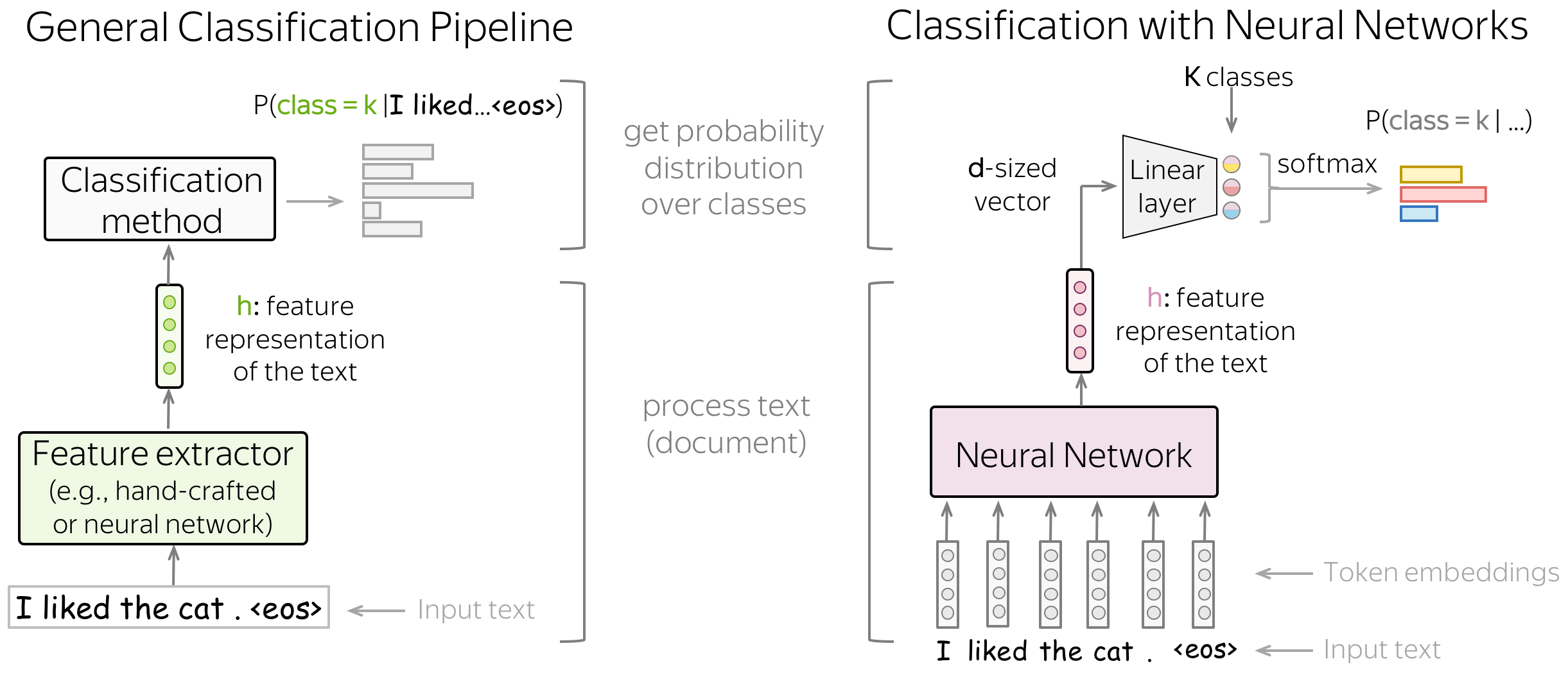When dealing with neural networks, we can think about the classification part (i.e., how to get class probabilities from a vector representation of a text) in a very simple way.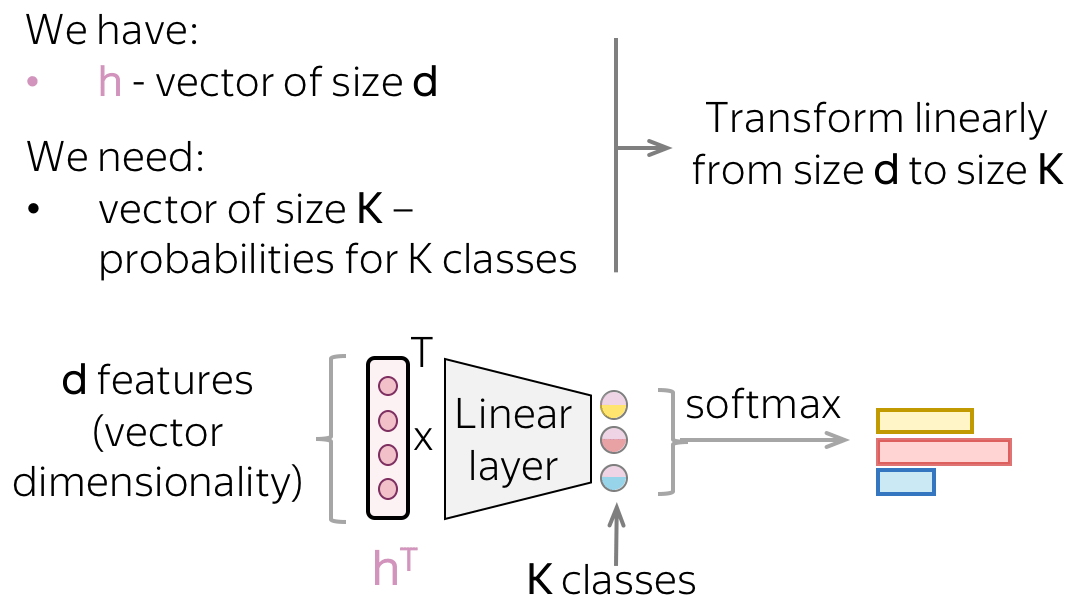Vector representation of a text has some dimensionality $$d$$, but in the end, we need a vector of size $$K$$ (probabilities for $$K$$ classes). To get a $$K$$-sized vector from a $$d$$-sized, we can use a linear layer. Once we have a $$K$$-sized vector, all is left is to apply the softmax operation to convert the raw numbers into class probabilities.

### Classification Part: This is Logistic Regression!

Let us look closer to the neural network classifier. The way we use vector representation of the input text is exactly the same as we did with logistic regression: we weigh features according to feature weights for each class. The only difference from logistic regression is where the features come from: they are either defined manually (as we did before) or obtained by a neural network.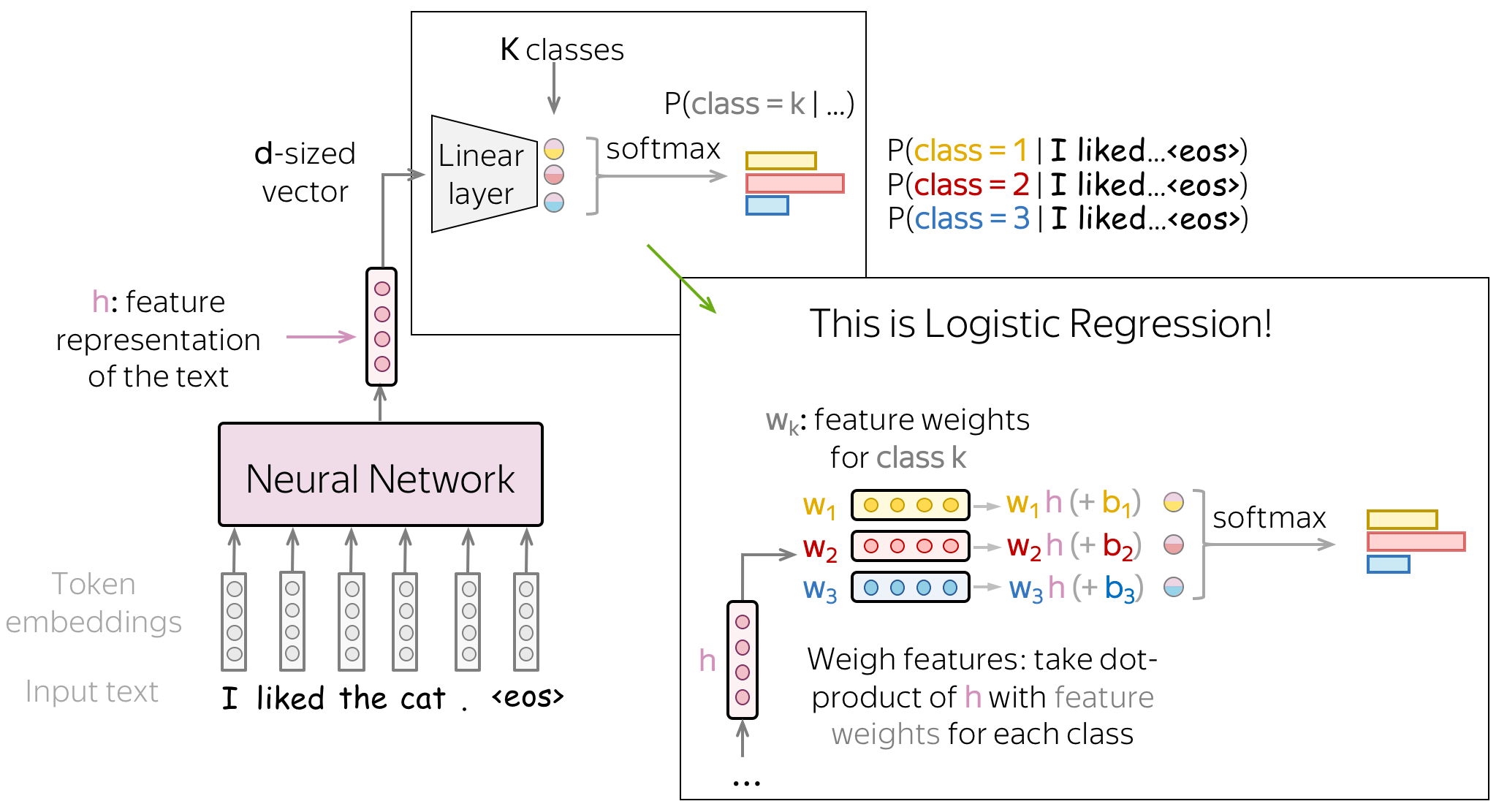### Intuition: Text Representation Points in the Direction of Class Representation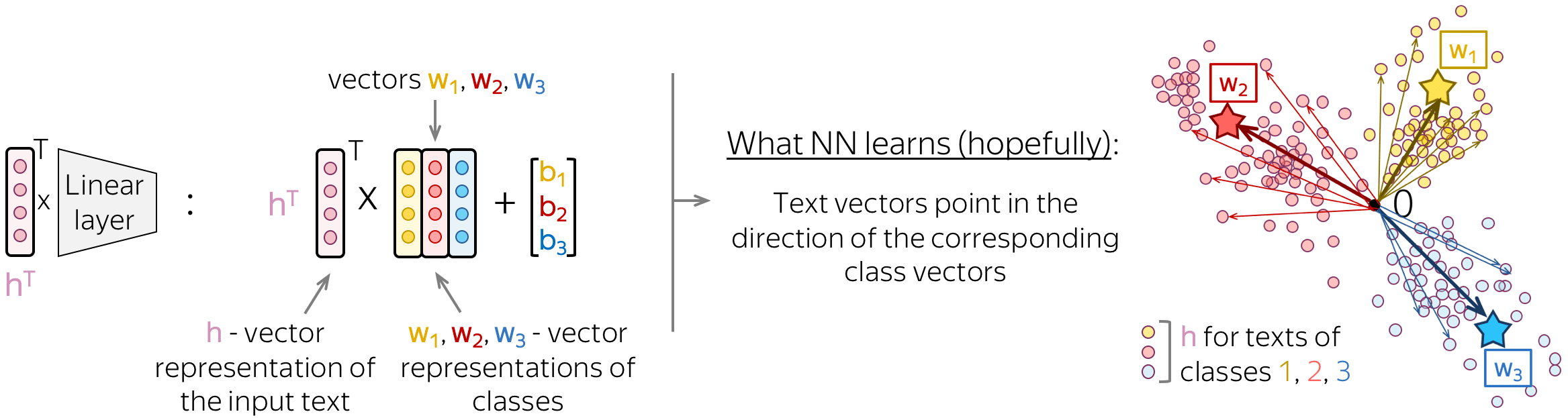If we look at this final linear layer more closely, we will see that the columns of its matrix are vectors $$w_i$$. These vectors can be thought of as vector representations of classes. A good neural network will learn to represent input texts in such a way that text vectors will point in the direction of the corresponding class vectors.

## Training and the Cross-Entropy Loss

Neural classifiers are trained to predict probability distributions over classes. Intuitively, at each step we maximize the probability a model assigns to the correct class.

The standard loss function is the cross-entropy loss. Cross-entropy loss for the target probability distribution $$p^{\ast} = (0, \dots, 0, 1, 0, \dots)$$ (1 for the target label, 0 for the rest) and the predicted by the model distribution $$p=(p_1, \dots, p_K), p_i=p(i|x)$$: $Loss(p^{\ast}, p^{})= - p^{\ast} \log(p) = -\sum\limits_{i=1}^{K}p_i^{\ast} \log(p_i).$ Since only one of $$p_i^{\ast}$$ is non-zero (1 for the target label $$k$$, 0 for the rest), we will get $$Loss(p^{\ast}, p) = -\log(p_{k})=-\log(p(k| x)).$$ Look at the illustration for one training example.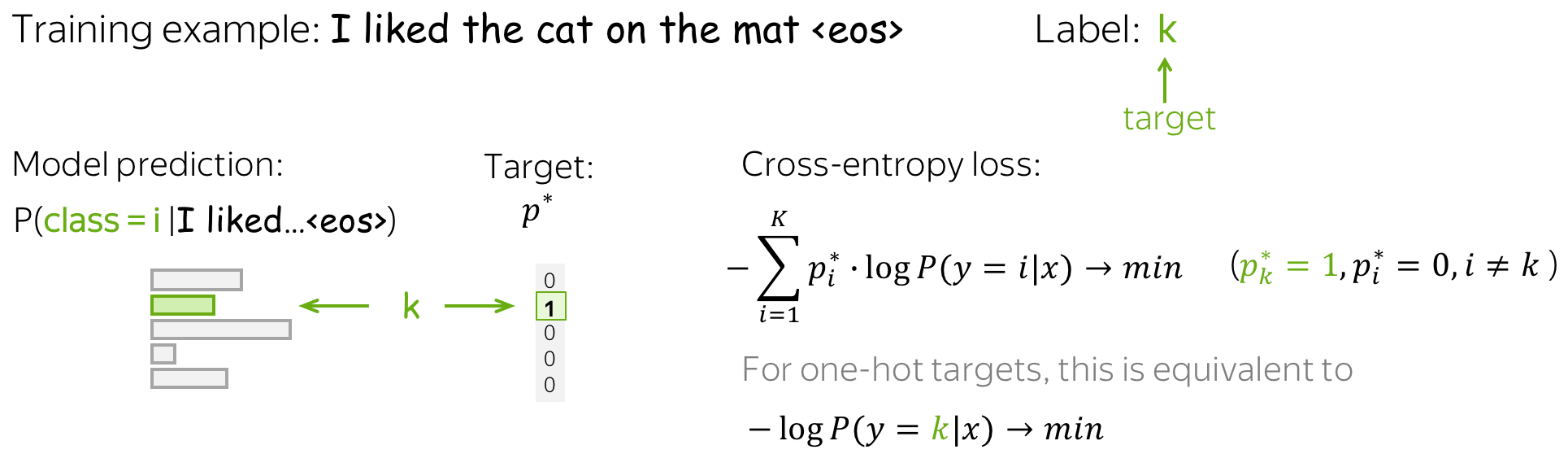In training, we gradually improve model weights during multiple iterations over the data: we iterate over training examples (or batches of examples) and make gradient updates. At each step, we maximize the probability a model assigns to the correct class. At the same time, we minimize sum of the probabilities of incorrect classes: since sum of all probabilities is constant, by increasing one probability we decrease sum of all the rest (Lena: Here I usually imagine a bunch of kittens eating from the same bowl: one kitten always eats at the expense of the others).

Look at the illustration of the training process.

### Recap: This is equivalent to maximizing the data likelihood

Do not forget that when talking about MaxEnt classifier (logistic regression), we showed that minimizing cross-entropy is equivalent to maximizing the data likelihood. Therefore, here we are also trying to get the Maximum Likelihood Estimate (MLE) of model parameters.

## Models for Text Classification

We need a model that can produce a fixed-sized vector for inputs of different lengths.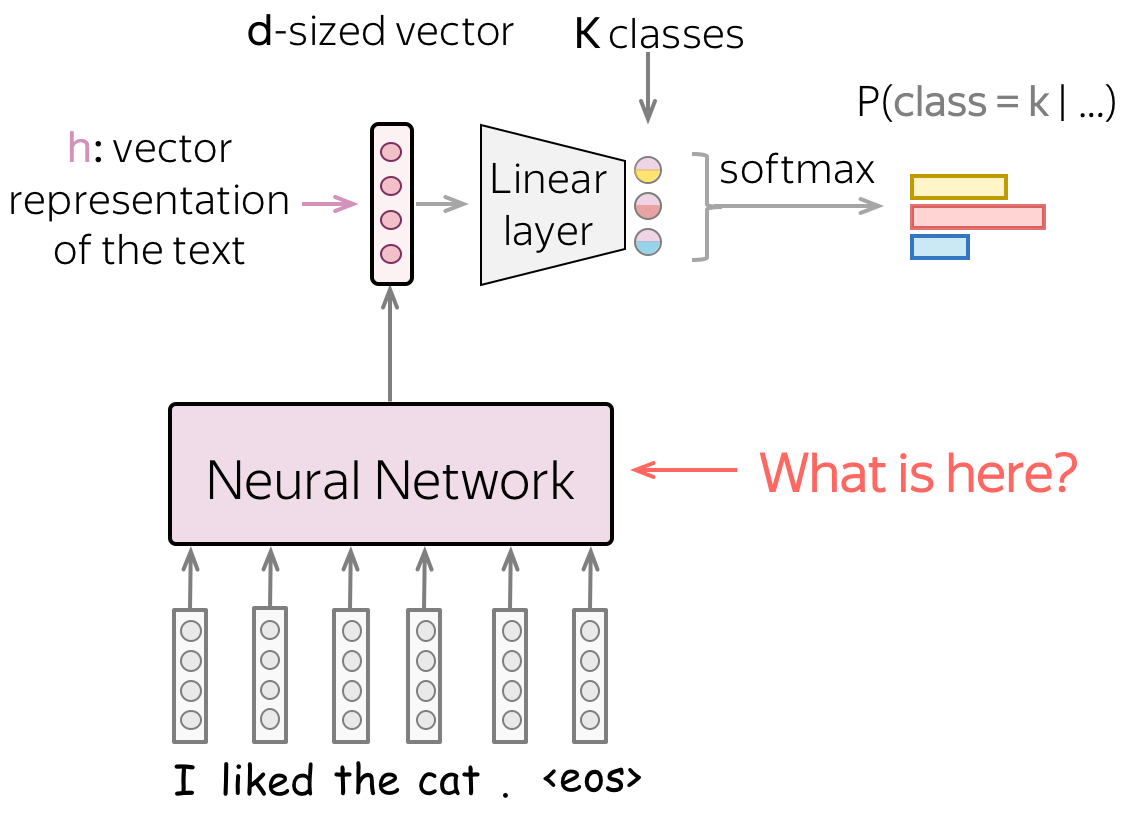In this part, we will look at different ways to get a vector representation of an input text using neural networks. Note that while input texts can have different lengths, the vector representation of a text has to have a fixed size: otherwise, a network will not "work".

We begin with the simplest approaches which use only word embeddings (without adding a model on top of that). Then we look at recurrent and convolutional networks.

Lena: A bit later in the course, you will learn about Transformers and the most recent classification techniques using large pretrained models.

## Basics: Bag of Embeddings (BOE) and Weighted BOE

The simplest you can do is use only word embeddings without any neural network on top of that. To get vector representation of a text, we can either sum all token embeddings (Bag of Embeddings) or use a weighted sum of these embeddings (with weights, for example, being tf-idf or something else).

Bag of Embeddings (ideally, along with Naive Bayes) should be a baseline for any model with a neural network: if you can't do better than that, it's not worth using NNs at all. This can be the case if you don't have much data.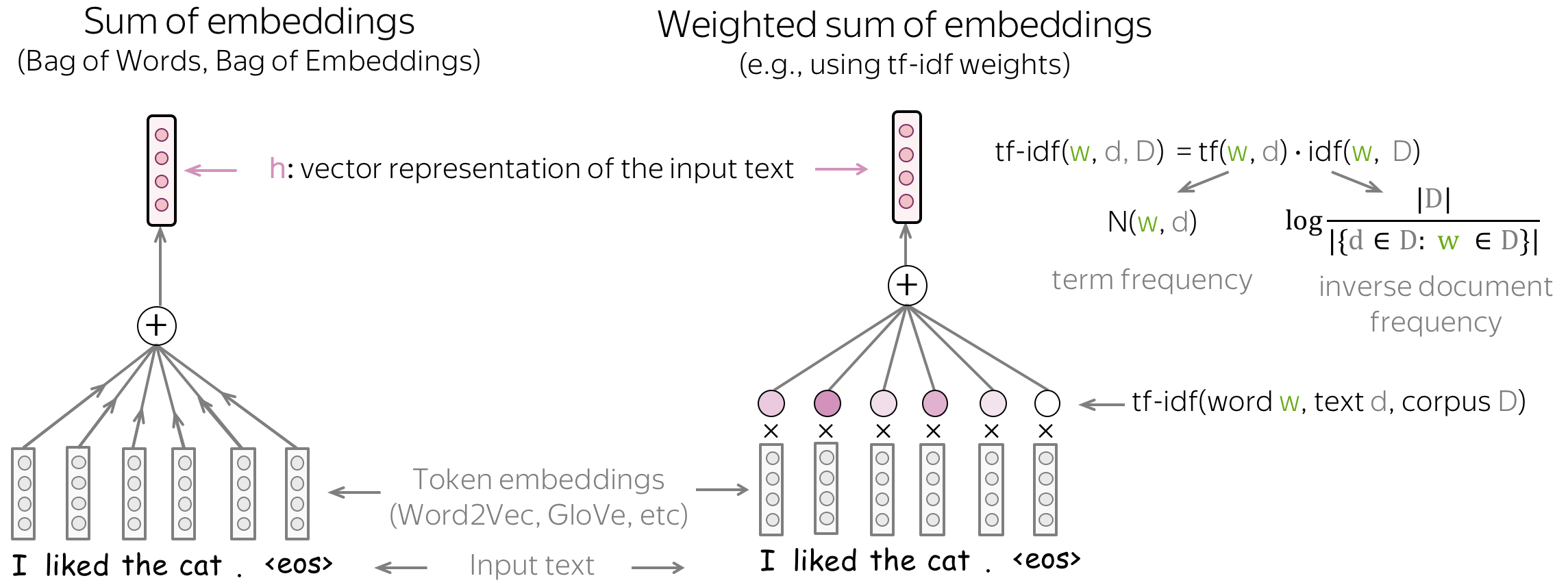While Bag of Embeddings (BOE) is sometimes called Bag of Words (BOW), note that these two are very different. BOE is the sum of embeddings and BOW is the sum of one-hot vectors: BOE knows a lot more about language. The pretrained embeddings (e.g., Word2Vec or GloVe) understand similarity between words. For example, awesome, brilliant, great will be represented with unrelated features in BOW but similar word vectors in BOE.

Note also that to use a weighted sum of embeddings, you need to come up with a way to get weights. However, this is exactly what we wanted to avoid by using neural networks: we don't want to introduce manual features, but rather let a network to learn useful patterns.

### Bag of Embeddings as Features for SVM

You can use SVM on top of BOE! The only difference from SVMs in classical approaches (on top of bag-of-words and bag-of-ngrams) if the choice of a kernel: here the RBF kernel is better.

## Models: Recurrent (RNN/LSTM/etc)

Recurrent networks are a natural way to process text in a sense that, similar to humans, they "read" a sequence of tokens one by one and process the information. Hopefully, at each step the network will "remember" everything it has read before.

### Basics: Recurrent Neural Networks

#### •RNN cell

At each step, a recurrent network receives a new input vector (e.g., token embedding) and the previous network state (which, hopefully, encodes all previous information). Using this input, the RNN cell computes the new state which it gives as output. This new state now contains information about both current input and the information from previous steps.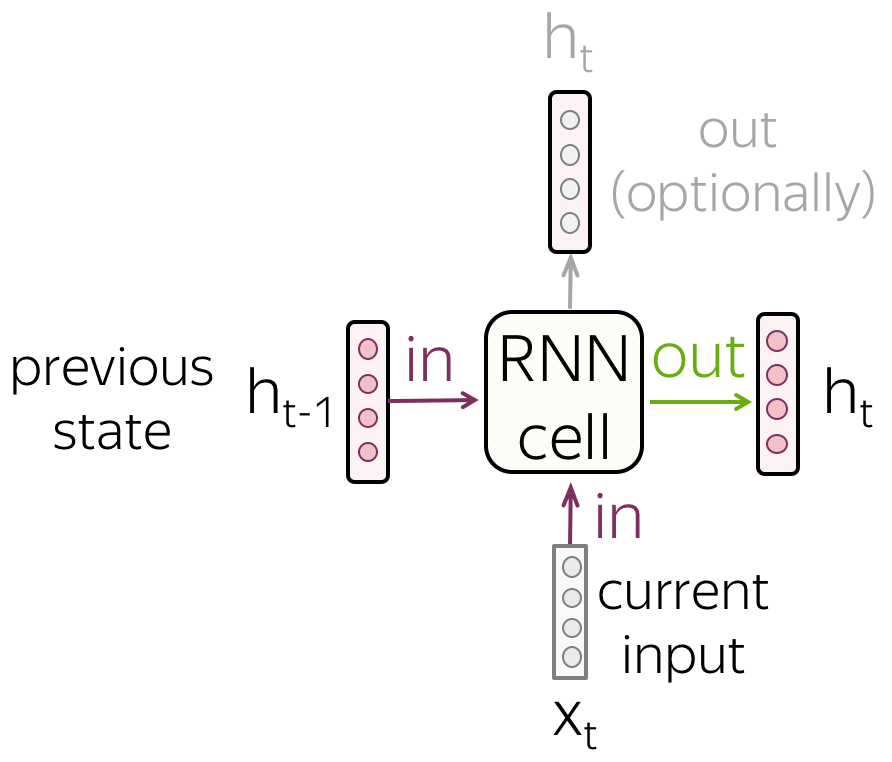#### •RNN reads a sequence of tokens

Look at the illustration: RNN reads a text token by token, at each step using a new token embedding and the previous state.

Note that the RNN cell is the same at each step!

#### •Vanilla RNN

The simplest recurrent network, Vanilla RNN, transforms $$h_{t-1}$$ and $$x_t$$ linearly, then applies a non-linearity (most often, the $$\tanh$$ function): $h_t = \tanh(h_{t-1}W_h + x_tW_t).$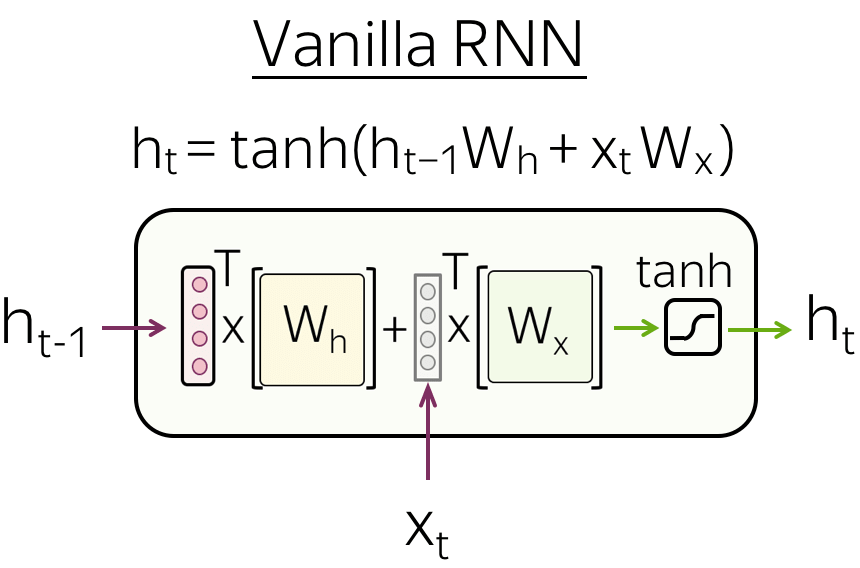Vanilla RNNs suffer from the vanishing and exploding gradients problem. To alleviate this problem, more complex recurrent cells (e.g., LSTM, GRU, etc) perform several operations on the input and use gates. For more details of RNN basics, look at the Colah's blog post.

### Recurrent Neural Networks for Text Classification

Here we (finally!) look at how we can use recurrent models for text classification. Everything you will see here will apply to all recurrent cells, and by "RNN" in this part I refer to recurrent cells in general (e.g. vanilla RNN, LSTM, GRU, etc).

Let us recall what we need:

We need a model that can produce a fixed-sized vector for inputs of different lengths.

#### •Simple: read a text, take the final state

The most simple recurrent model is a one-layer RNN network. In this network, we have to take the state which knows more about input text. Therefore, we have to use the last state - only this state saw all input tokens.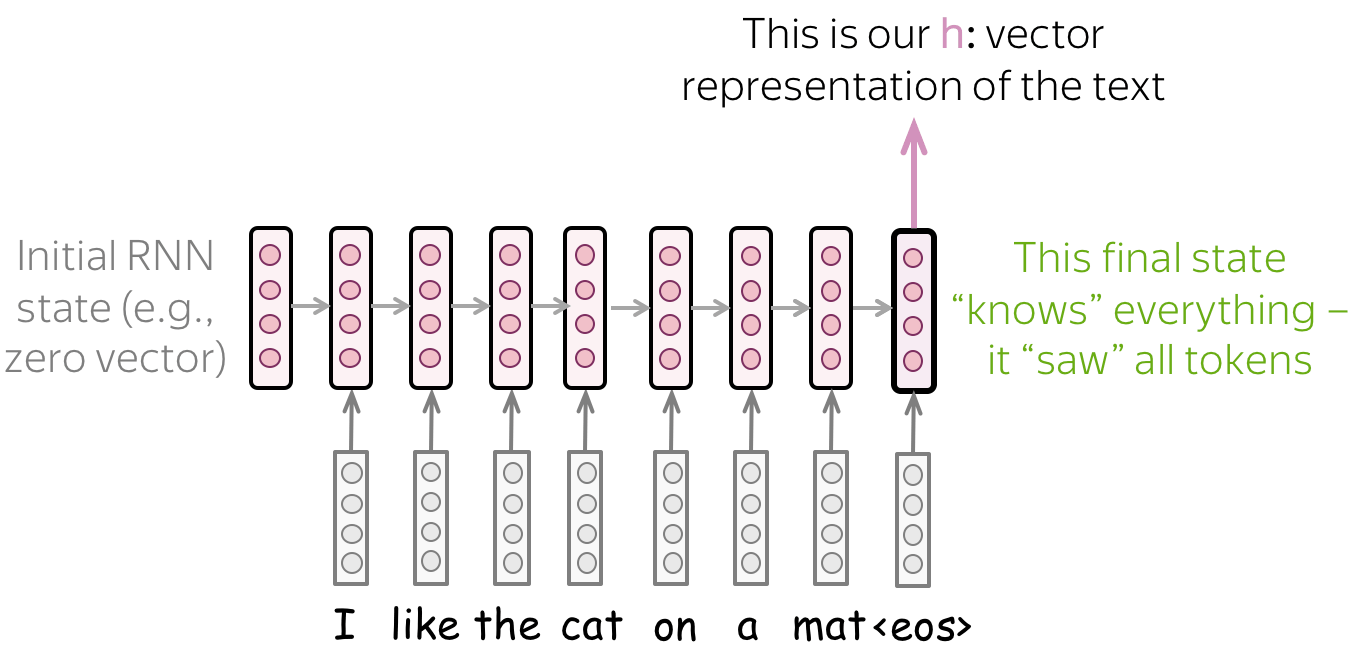#### •Multiple layers: feed the states from one RNN to the next one

To get a better text representation, you can stack multiple layers. In this case, inputs for the higher RNN are representations coming from the previous layer.

The main hypothesis is that with several layers, lower layers will catch local phenomena (e.g., phrases), while higher layers will be able to learn more high-level things (e.g., topic).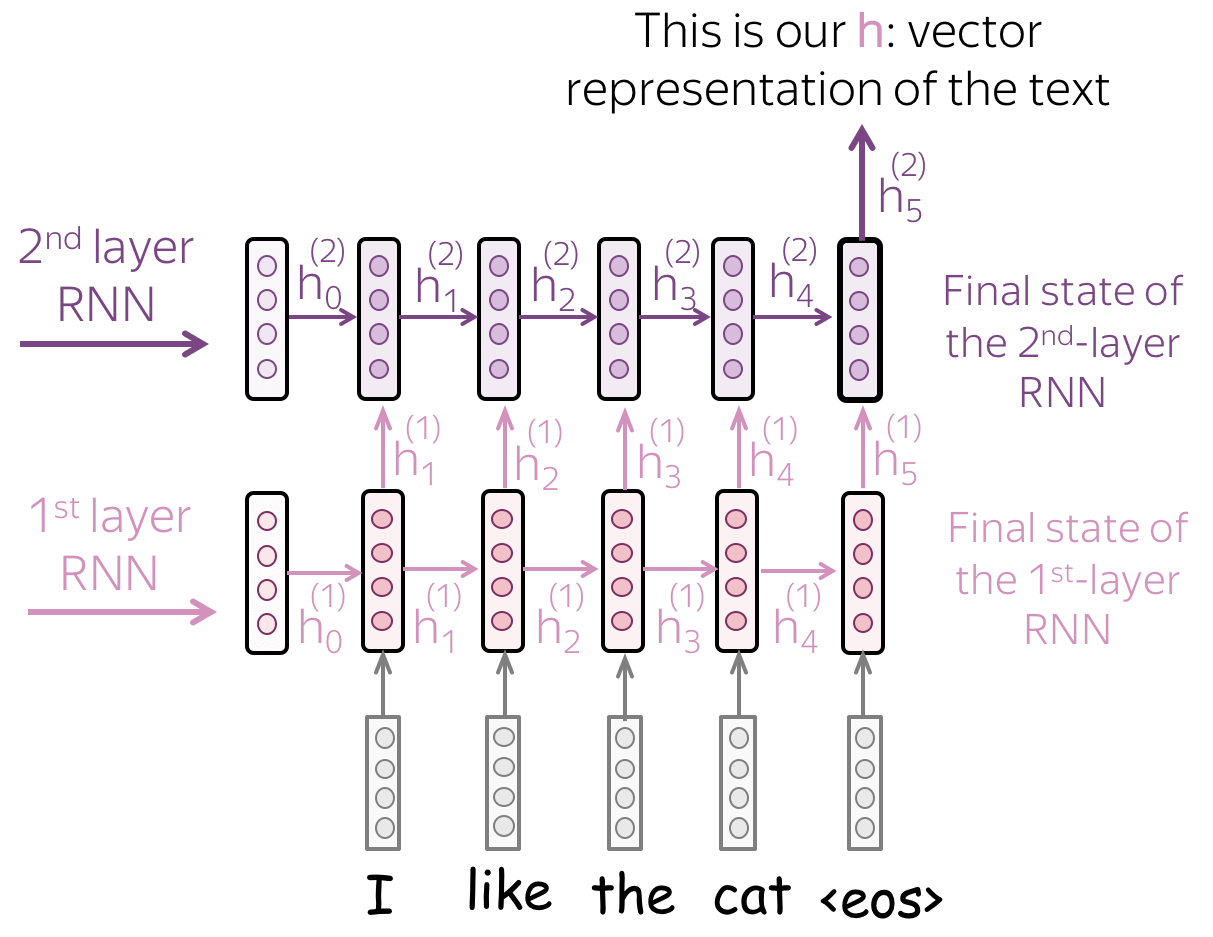#### •Bidirectional: use final states from forward and backward RNNs.

Previous approaches may have a problem: the last state can easily "forget" earlier tokens. Even strong models such as LSTMs can still suffer from that!

To avoid this, we can use two RNNs: forward, which reads input from left to right, and backward, which reads input from right to left. Then we can use the final states from both models: one will better remember the final part of a text, another - the beginning. These states can be concatenated, or summed, or something else - it's your choice!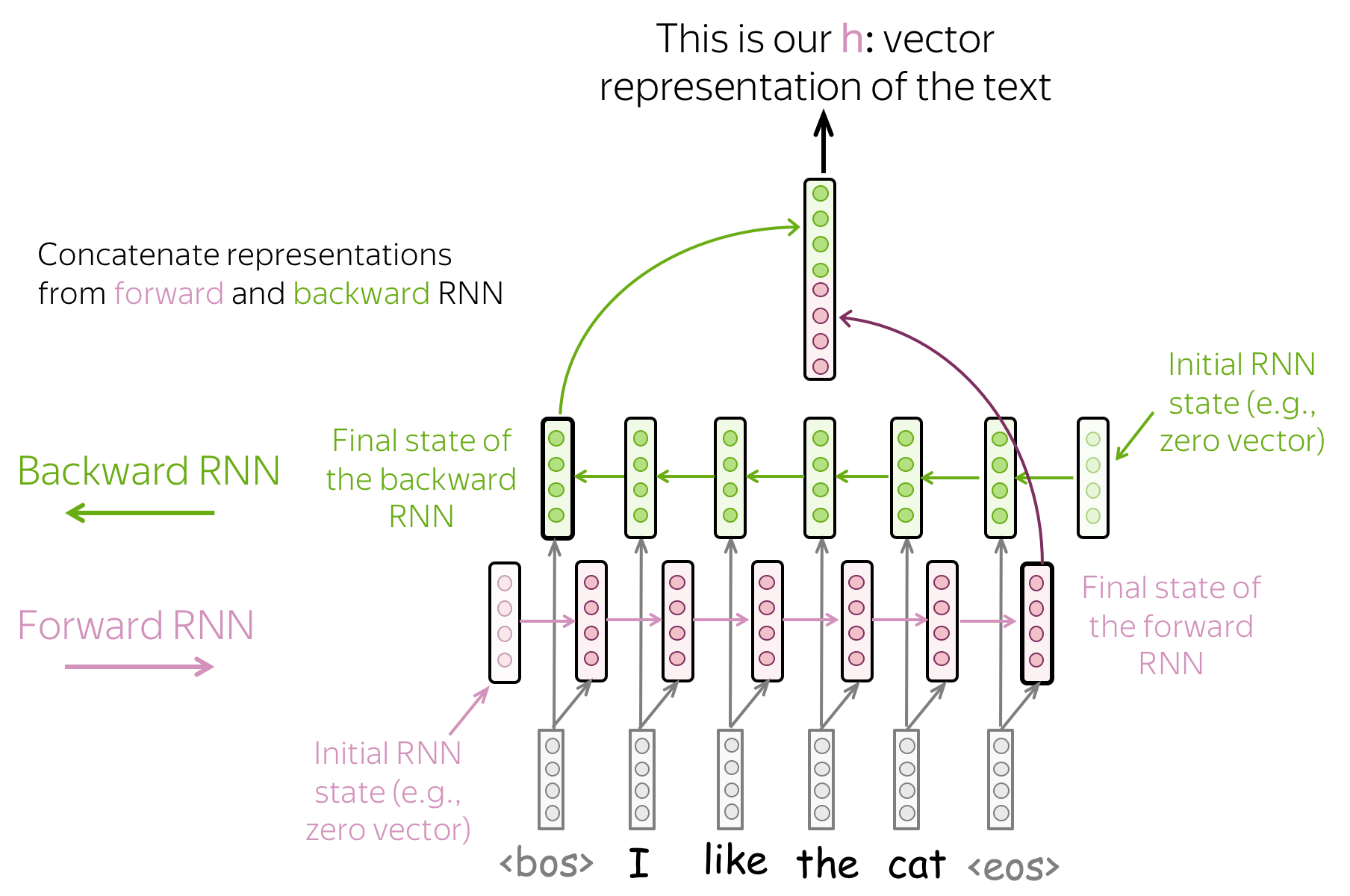#### •Combinations: do everything you want!

You can combine the ideas above. For example, in a multi-layered network, some layers can go in the opposite direction, etc.

## Models: Convolutional (CNN)

The detailed description of convolutional models in general is in Convolutional Models Supplementary. In this part, we consider only convolutions for text classification.

#### Convolutions for Images and Translation Invariance

Convolutional networks were originally developed for computer vision tasks. Therefore, let's first understand the intuition behind convolutional models for images.

Imagine we want to classify an image into several classes, e.g. cat, dog, airplane, etc. In this case, if you find a cat on an image, you don't care where on the image this cat is: you care only that it is there somewhere.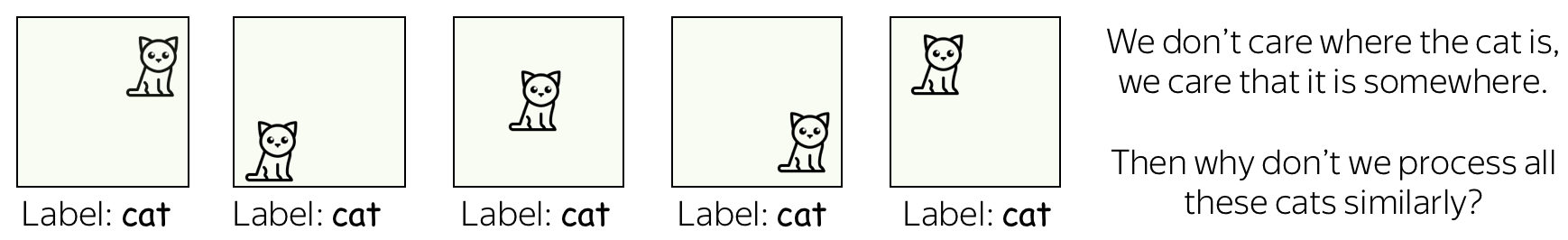Convolutional networks apply the same operation to small parts of an image: this is how they extract features. Each operation is looking for a match with a pattern, and a network learns which patterns are useful. With a lot of layers, the learned patterns become more and more complicated: from lines in the early layers to very complicated patterns (e.g., the whole cat or dog) on the upper ones. You can look at the examples in the Analysis and Interpretability section.

This property is called translation invariance: translation because we are talking about shifts in space, invariance because we want it to not matter.

The illustration is adapted from the one taken from this cool repo.

#### Convolutions for Text

Well, for images it's all clear: e.g. we want to be able to move a cat because we don't care where the cat is. But what about texts? At first glance, this is not so straightforward: we can not move phrases easily - the meaning will change or we will get something that does not make much sense.

However, there are some applications where we can think of the same intuition. Let's imagine that we want to classify texts, but not cats/dogs as in images, but positive/negative sentiment. Then there are some words and phrases which could be very informative "clues" (e.g. it's been great, bored to death, absolutely amazing, the best ever, etc), and others which are not important at all. We don't care much where in a text we saw bored to death to understand the sentiment, right?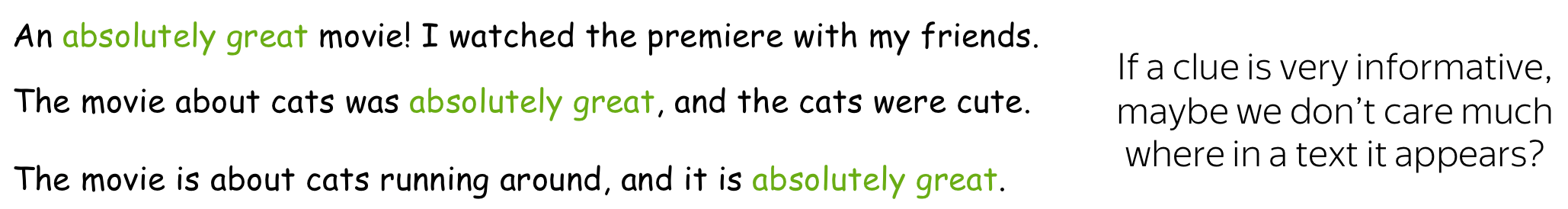#### A Typical Model: Convolution+Pooling Blocks

Following the intuition above, we want to detect some patterns, but we don't care much where exactly these patterns are. This behavior is implemented with two layers:

• convolution: finds matches with patterns (as the cat head we saw above);
• pooling: aggregates these matches over positions (either locally or globally).

A typical convolutional model for text classification is shown on the figure. To get a vector representation of an input text, a convolutional layer is applied to word embedding, which is followed by a non-linearity (usually ReLU) and a pooling operation. The way this representation is used for classification is similar to other networks.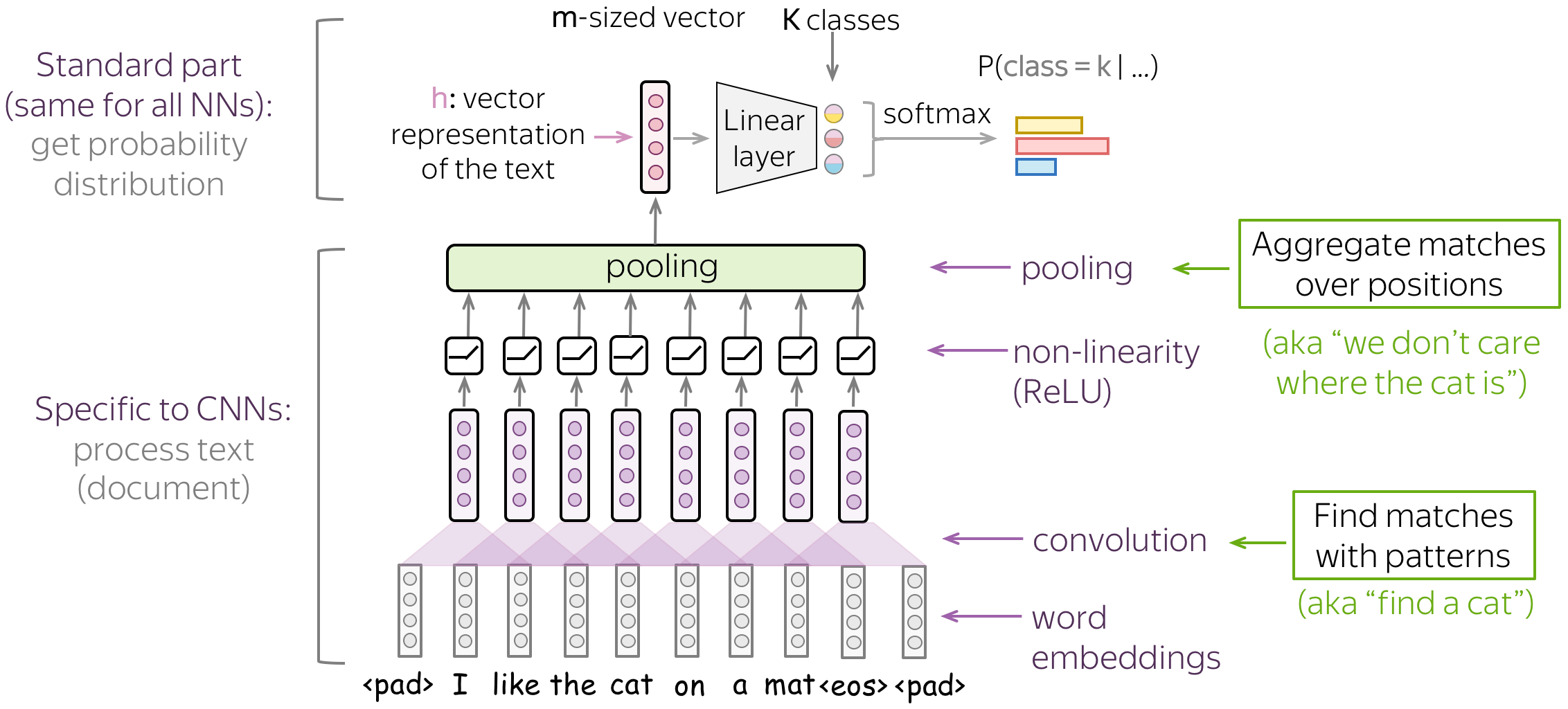In the following, we discuss in detail the main building blocks, convolution and pooling, then consider modeling modifications.

### Basics: Convolution Layer for Text

Convolutional Neural Networks were initially developed for computer vision tasks, e.g. classification of images (cats vs dogs, etc). The idea of a convolution is to go over an image with a sliding window and to apply the same operation, convolution filter, to each window.

The illustration (taken from this cool repo) shows this process for one filter: the bottom is the input image, the top is the filter output. Since an image has two dimensions (width and height), the convolution is two-dimensional.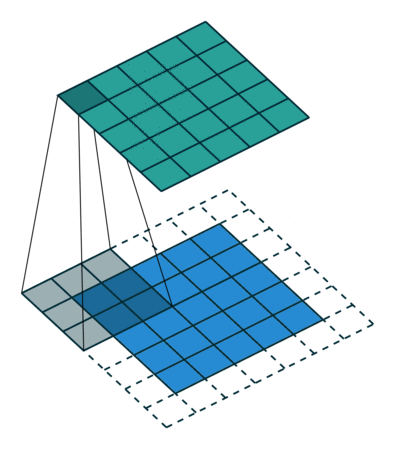Convolution filter for images. The illustration is from this cool repo.

Differently from images, texts have only one dimension: here a convolution is one-dimensional: look at the illustration.

Convolution filter for text.

#### Convolution is a Linear Operation Applied to Each Window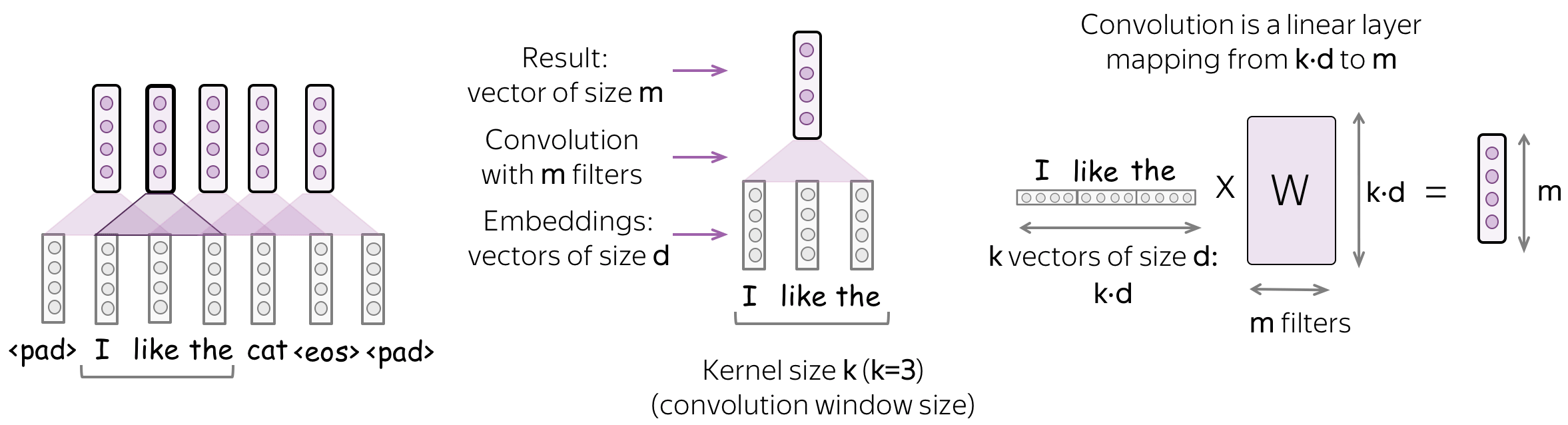A convolution is a linear layer (followed by a non-linearity) applied to each input window. Formally, let us assume that

• $$(x_1, \dots, x_n)$$ - representations of the input words, $$x_i\in \mathbb{R}^d$$;
• $$d$$ (input channels) - size of an input embedding;
• $$k$$ (kernel size) - the length of a convolution window (on the illustration, $$k=3$$);
• $$m$$ (output channels) - number of convolution filters (i.e., number of channels produced by the convolution).

Then a convolution is a linear layer $$W\in\mathbb{R}^{(k\cdot d)\times m}$$. For a $$k$$-sized window $$(x_i, \dots x_{i+k-1})$$, the convolution takes the concatenation of these vectors $u_i = [x_i, \dots x_{i+k-1}]\in\mathbb{R}^{k\cdot d}$ and multiplies by the convolution matrix: $F_i = u_i \times W.$ A convolution goes over an input with a sliding window and applies the same linear transformation to each window.

#### Intuition: Each Filter Extracts a Feature

Intuitively, each filter in a convolution extracts a feature.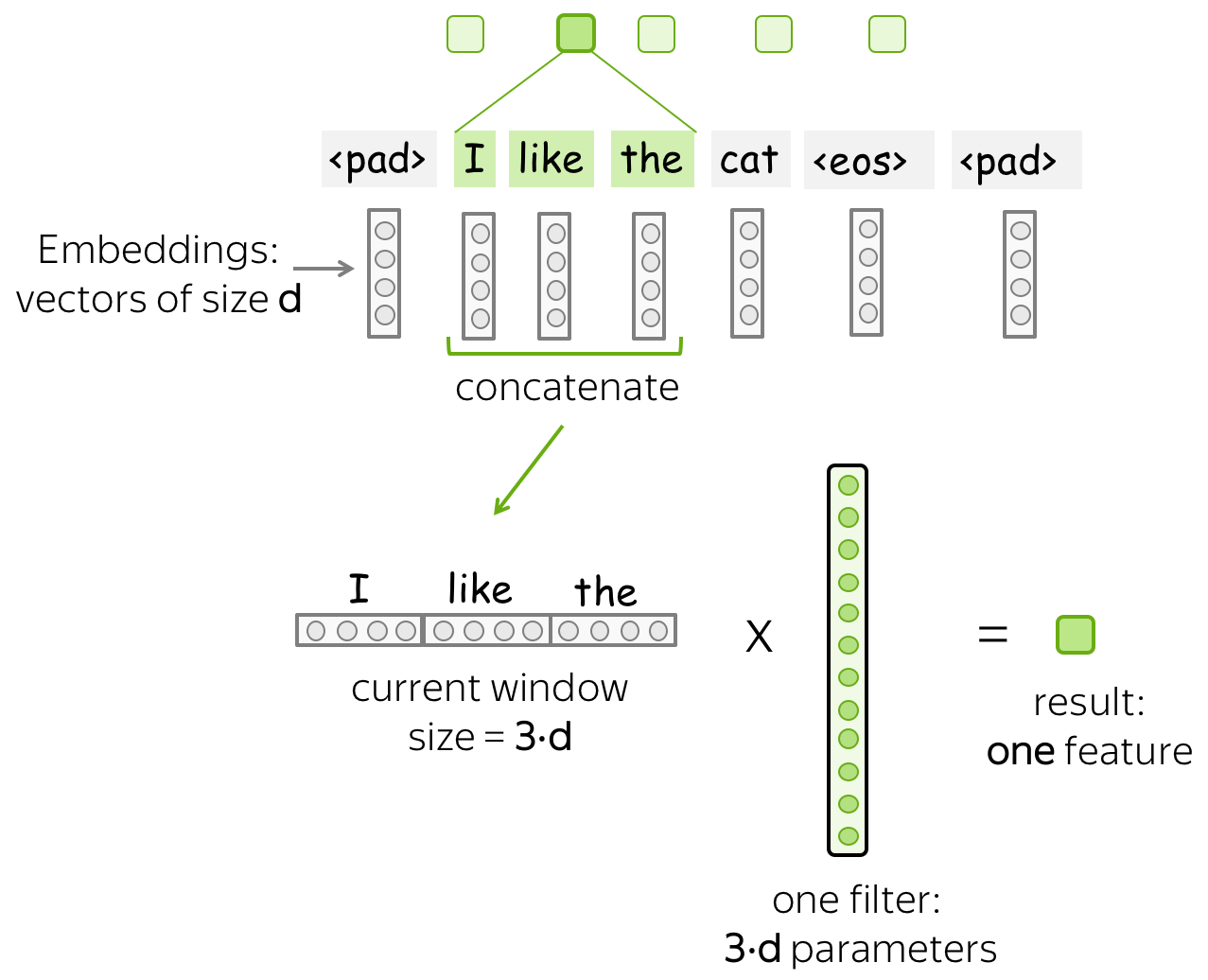### •One filter - one feature extractor

A filter takes vector representations in a current window and transforms them linearly into a single feature. Formally, for a window $$u_i = [x_i, \dots x_{i+k-1}]\in\mathbb{R}^{k\cdot d}$$ a filter $$f\in\mathbb{R}^{k\cdot d}$$ computes dot product: $F_i^{(f)} = (f, u_i).$ The number $$F_i^{(f)}$$ (the extracted "feature") is a result of applying the filter $$f$$ to the window $$(x_i, \dots x_{i+k-1})$$.

#### •m filters: m feature extractors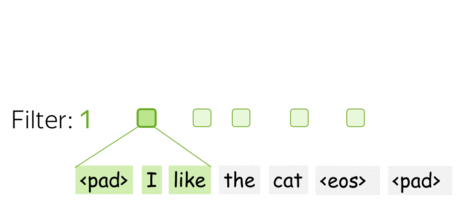One filter extracts a single feature. Usually, we want many features: for this, we have to take several filters. Each filter reads an input text and extracts a different feature - look at the illustration. The number of filters is the number of output features you want to get. With $$m$$ filters instead of one, the size of the convolutional layer we discussed above will become $$(k\cdot d)\times m$$.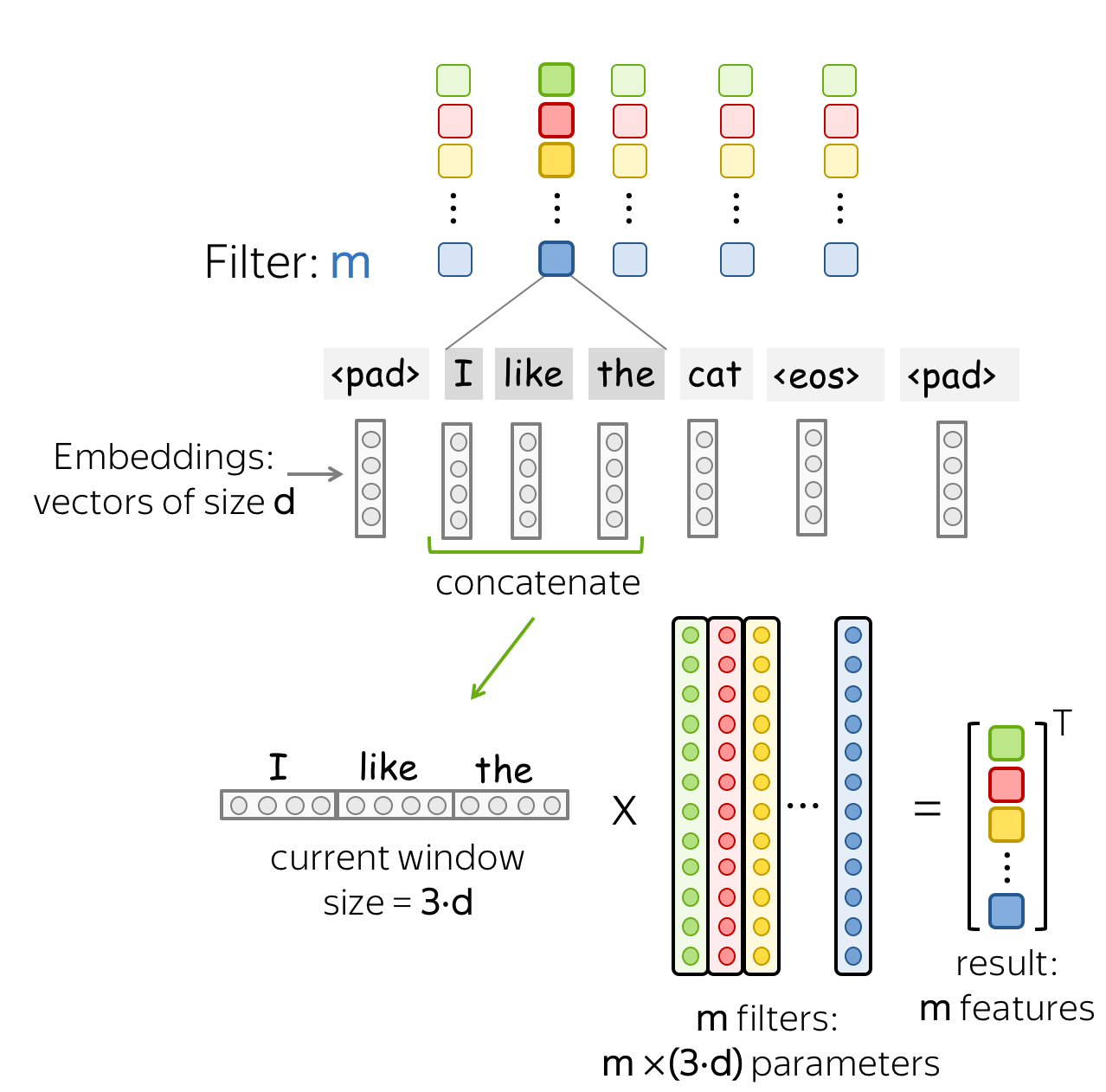This is done in parallel! Note that while I show you how a CNN "reads" a text, in practice these computations are done in parallel.

### Basics: Pooling Operation

After a convolution extracted $$m$$ features from each window, a pooling layer summarises the features in some region. Pooling layers are used to reduce the input dimension, and, therefore, to reduce the number of parameters used by the network.

#### •Max and Mean Pooling

The most popular is max-pooling: it takes maximum over each dimension, i.e. takes the maximum value of each feature.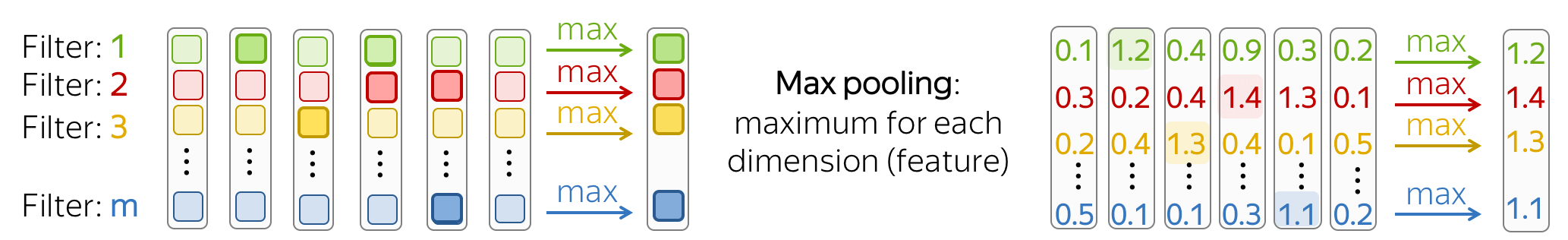Intuitively, each feature "fires" when it sees some pattern: a visual pattern in an image (line, texture, a cat's paw, etc) or a text pattern (e.g., a phrase). After a pooling operation, we have a vector saying which of these patterns occurred in the input.

Mean-pooling works similarly but computes mean over each feature instead of maximum.

#### •Pooling and Global Pooling

Similarly to convolution, pooling is applied to windows of several elements. Pooling also has the stride parameter, and the most common approach is to use pooling with non-overlapping windows. For this, you have to set the stride parameter the same as the pool size. Look at the illustration.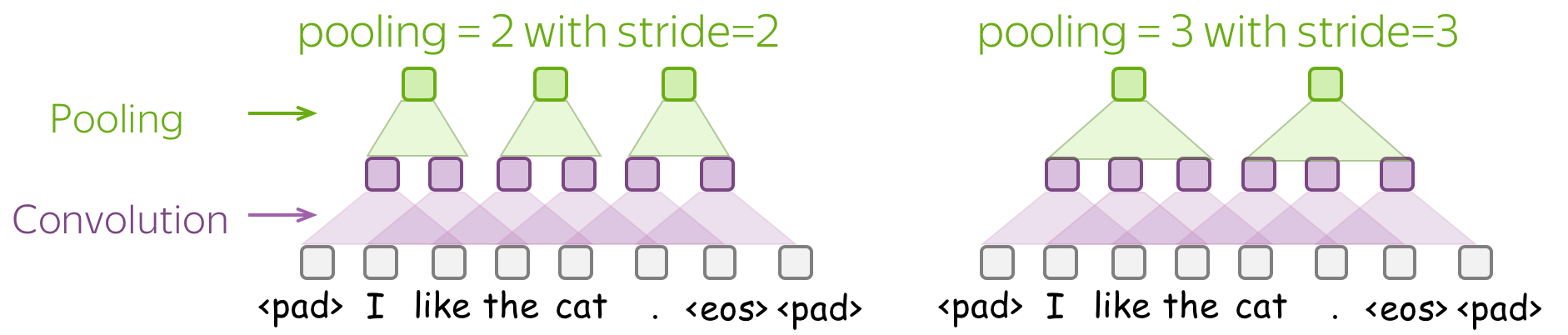The difference between pooling and global pooling is that pooling is applied over features in each window independently, while global pooling performs over the whole input. For texts, global pooling is often used to get a single vector representing the whole text; such global pooling is called max-over-time pooling, where the "time" axis goes from the first input token to the last.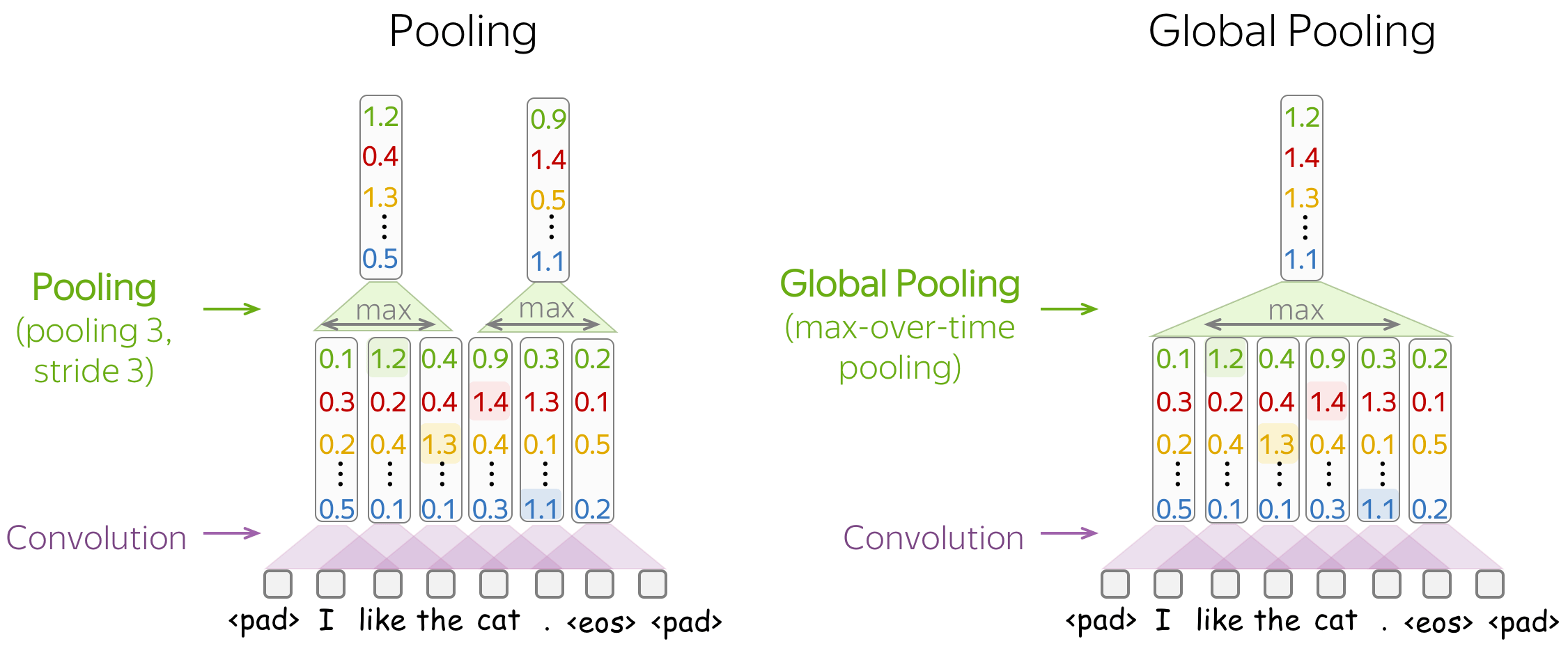### Convolutional Neural Networks for Text Classification

Now, when we understand how the convolution and pooling work, let's come to modeling modifications. First, let us recall what we need:

We need a model that can produce a fixed-sized vector for inputs of different lengths.

Therefore, we need to construct a convolutional model that represents a text as a single vector.

The basic convolutional model for text classification is shown on the figure. It is almost the same as we saw before: the only thing that's changed is that we specified the type of pooling used. Specifically, after the convolution, we use global-over-time pooling. This is the key operation: it allows to compress a text into a single vector. The model itself can be different, but at some point it has to use the global pooling to compress input in a single vector.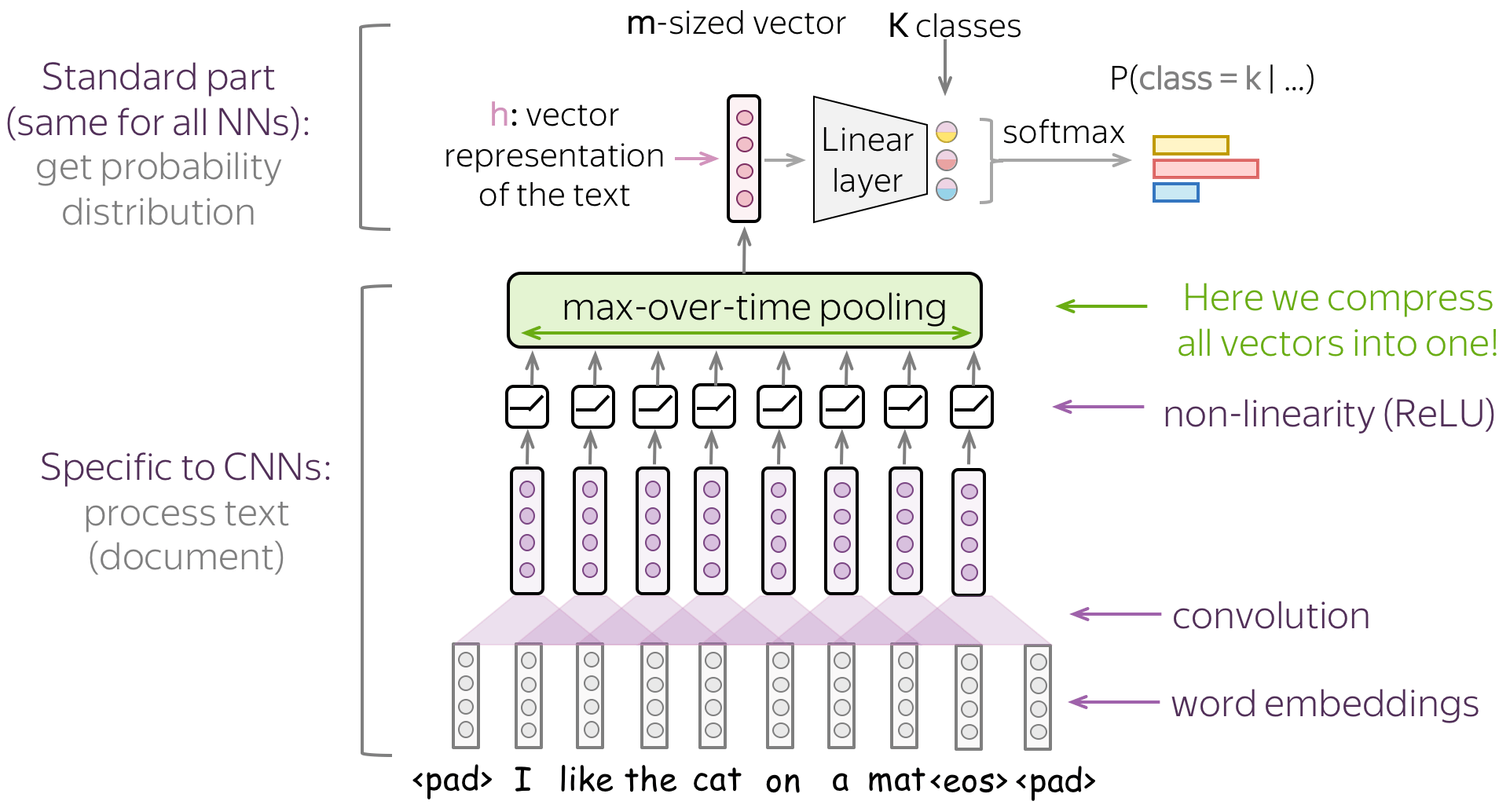#### •Several Convolutions with Different Kernel Sizes

Instead of picking one kernel size for your convolution, you can use several convolutions with different kernel sizes. The recipe is simple: apply each convolution to the data, add non-linearity and global pooling after each of them, then concatenate the results (on the illustration, non-linearity is omitted for simplicity). This is how you get vector representation of the data which is used for classification.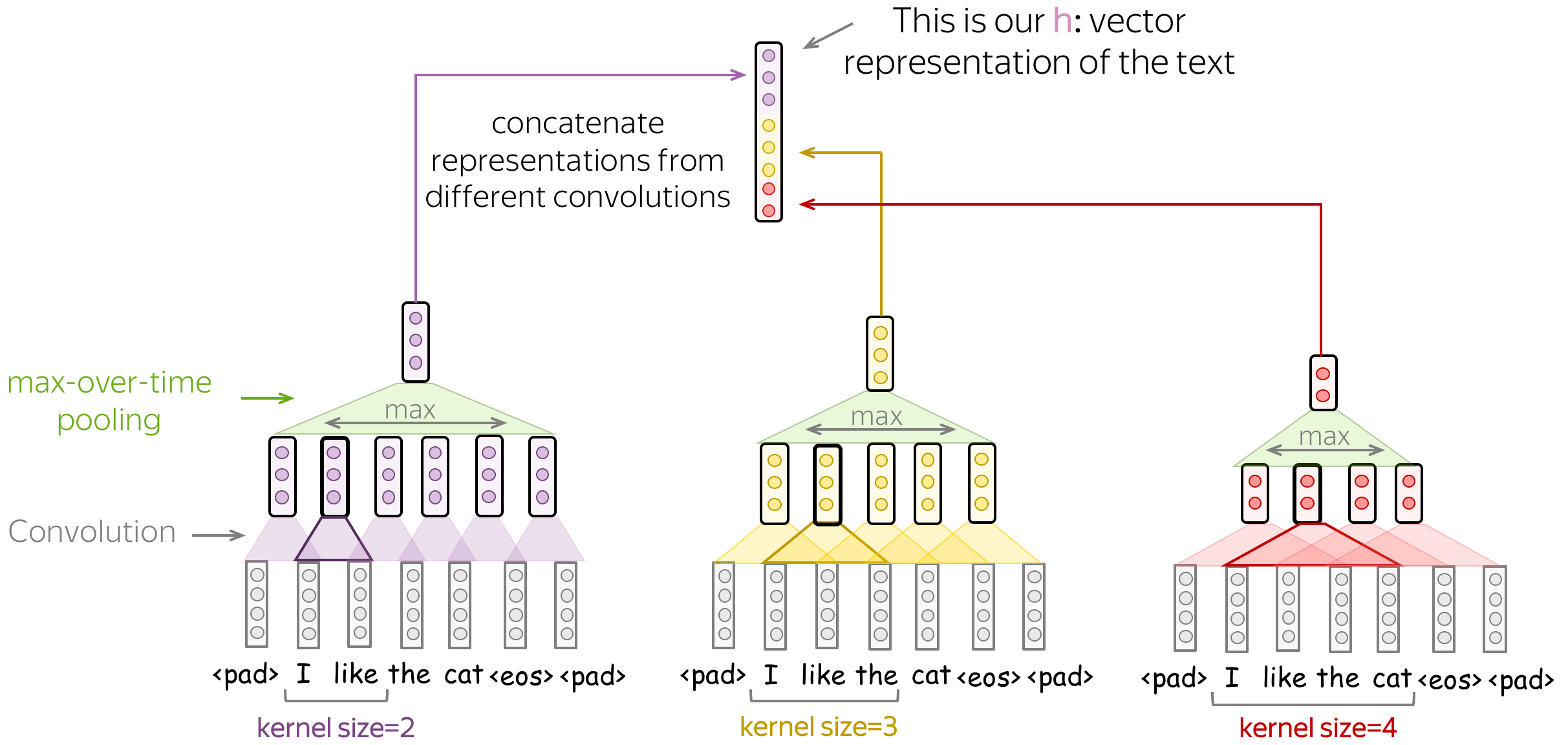This idea was used, among others, in the paper Convolutional Neural Networks for Sentence Classification and many follow-ups.

#### •Stack Several Blocks Convolution+Pooling

Instead of one layer, you can stack several blocks convolution+pooling on top of each other. After several blocks, you can apply another convolution, but with global pooling this time. Remember: you have to get a single fixed-sized vector - for this, you need global pooling.

Such multi-layered convolutions can be useful when your texts are very long; for example, if your model is character-level (as opposed to word-level).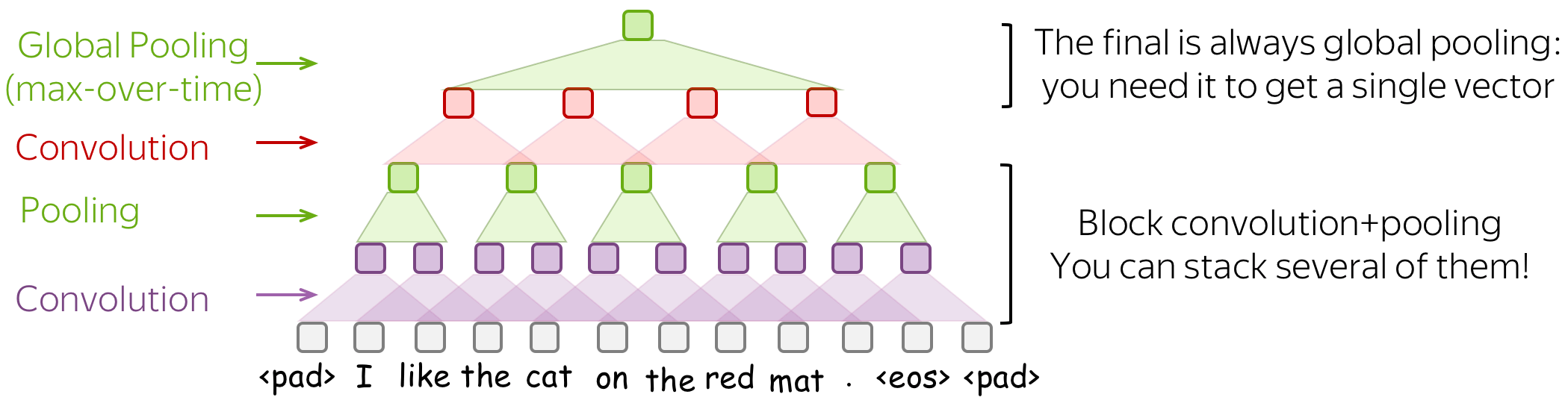This idea was used, among others, in the paper Character-level Convolutional Networks for Text Classification.

# Multi-Label Classification

Multi-label classification is different from the single-label problems we discussed before in that each input can have several correct labels. For example, a twit can have several hashtags, a user can have several topics of interest, etc.

For a multi-label problem, we need to change two things in the single-label pipeline we discussed before:

• model (how we evaluate class probabilities);
• loss function.

## Model: Softmax → Element-wise Sigmoid

After the last linear layer, we have $$K$$ values corresponding to the $$K$$ classes - these are the values we have to convert to class probabilities.

For single-label problems, we used softmax: it converts $$K$$ values into a probability distribution, i.e. sum of all probabilities is 1. It means that the classes share the same probability mass: if the probability of one class is high, other classes can not have large probability (Lena: Once again, imagine a bunch of kittens eating from the same bowl: one kitten always eats at the expense of the others).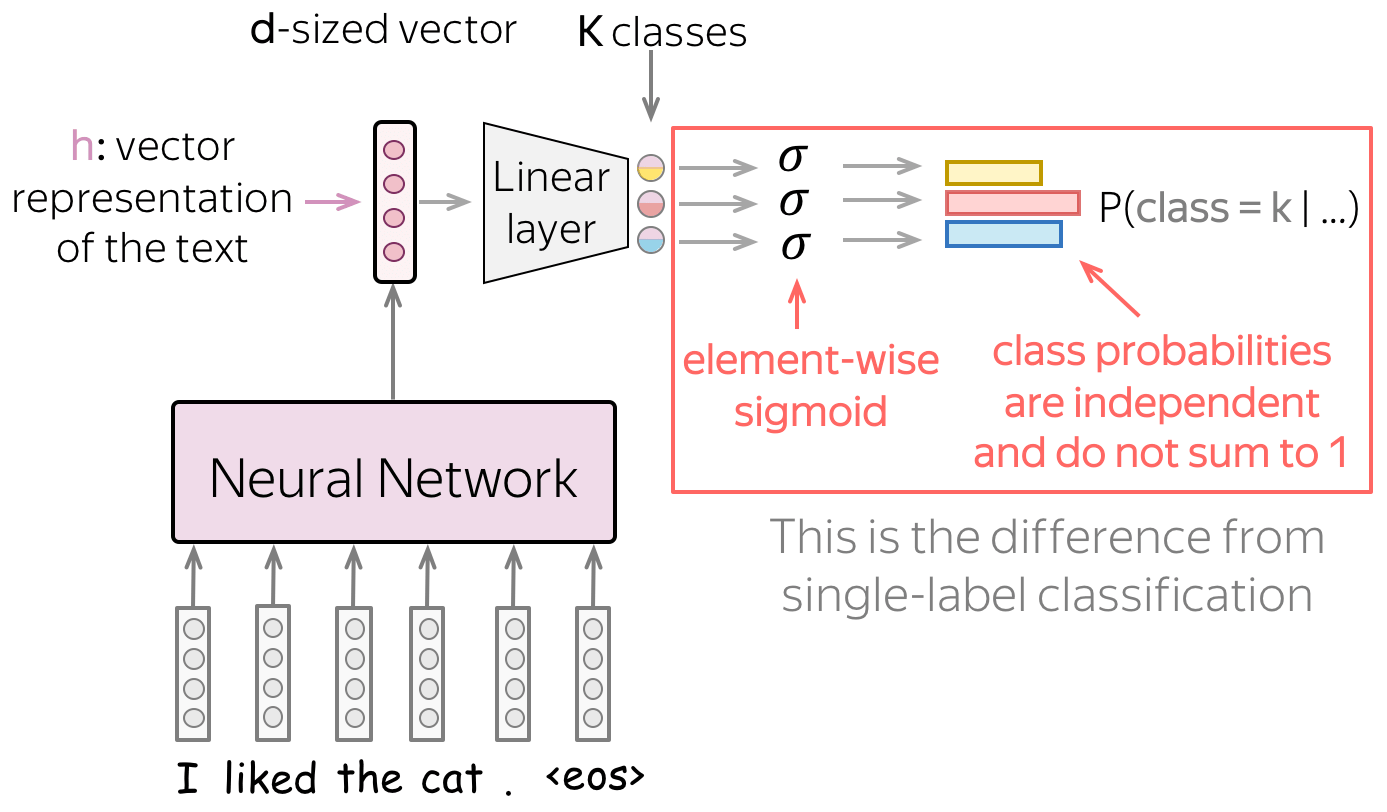For multi-label problems, we convert each of the $$K$$ values into a probability of the corresponding class independently from the others. Specifically, we apply the sigmoid function $$\sigma(x)=\frac{1}{1+e^{-x}}$$ to each of the $$K$$ values.

Intuitively, we can think of this as having $$K$$ independent binary classifiers that use the same text representation.

## Loss Function: Binary Cross-Entropy for Each Class

Loss function changes to enable multiple labels: for each class, we use the binary cross-entropy loss. Look at the illustration.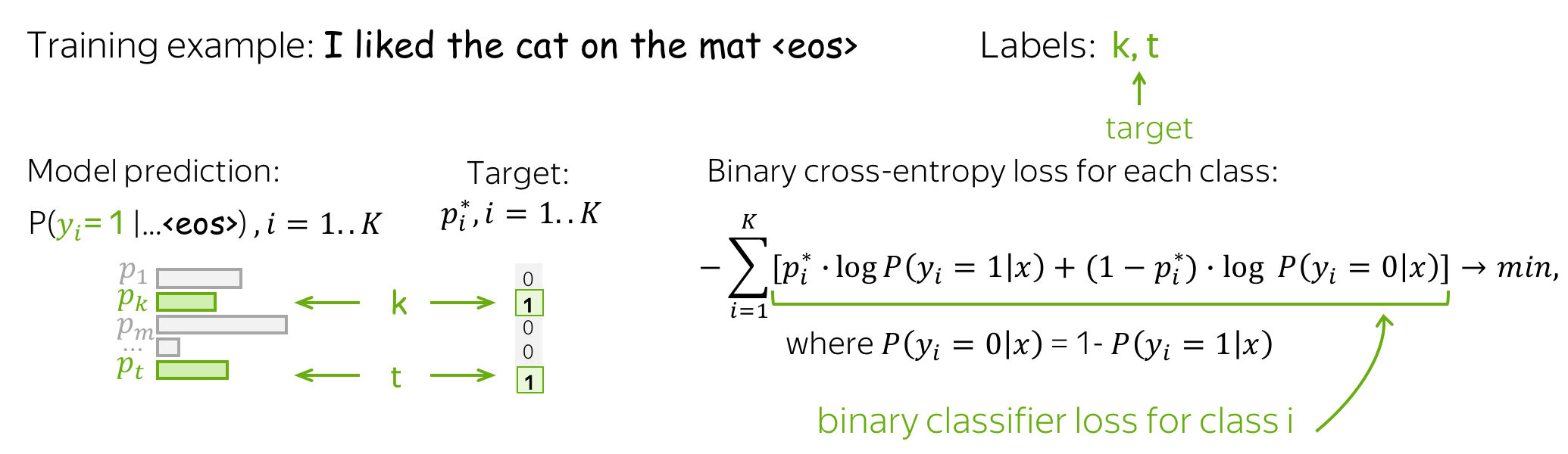# Practical Tips

## Word Embeddings: how to deal with them?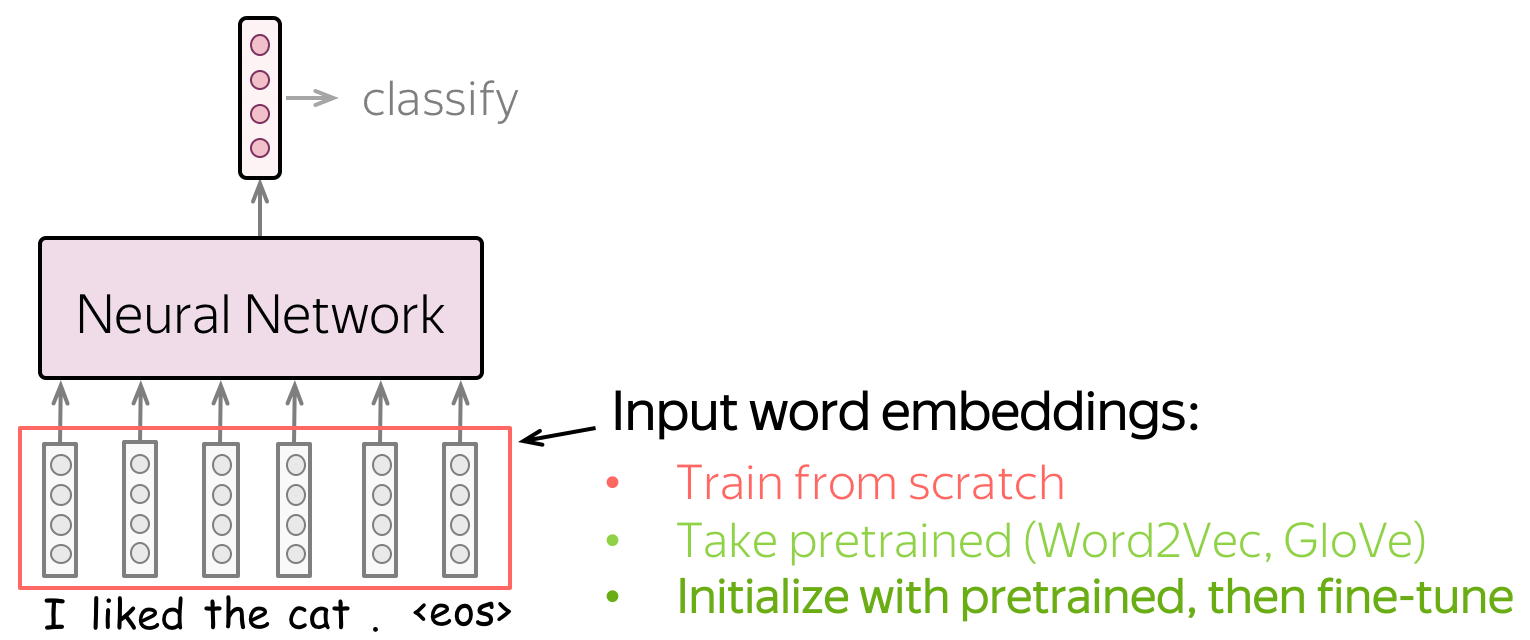Input for a network is represented by word embeddings. You have three options how to get these embeddings for your model:

• train from scratch as part of your model,
• take pretrained (Word2Vec, GloVe, etc) and fix them (use them as static vectors),
• initialize with pretrained embeddings and train them with the network ("fine-tune").

Let's think about these options by looking at the data a model can use. Training data for classification is labeled and task-specific, but labeled data is usually hard to get. Therefore, this corpus is likely to be not huge (at the very least), or not diverse, or both. On the contrary, training data for word embeddings is not labeled - plain texts are enough. Therefore, these datasets can be huge and diverse - a lot to learn from.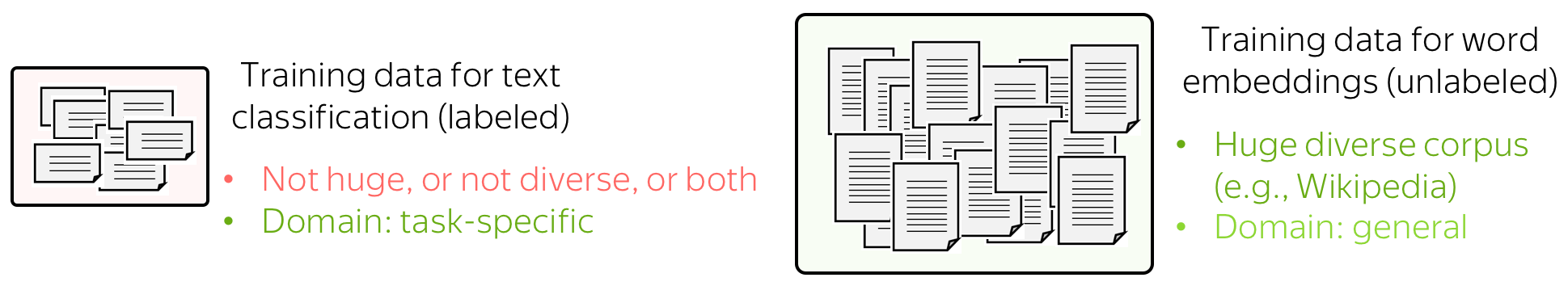Now let us think what a model will know depending on what we do with the embeddings. If the embeddings are trained from scratch, the model will "know" only the classification data - this may not be enough to learn relationships between words well. But if we use pretrained embeddings, they (and, therefore, the whole model) will know a huge corpus - they will learn a lot about the world. To adapt these embeddings to your task-specific data, you can fine-tune these embeddings by training them with the whole network - this can bring gains in the performance (not huge though).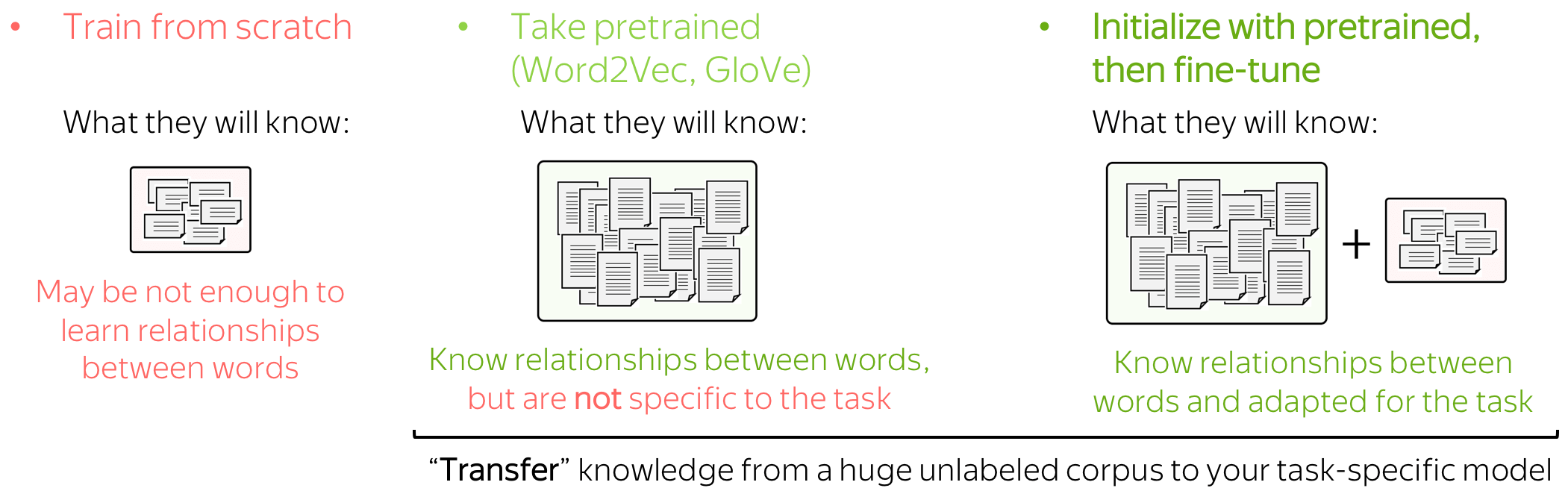When we use pretrained embeddings, this is an example of transfer learning: through the embeddings, we "transfer" the knowledge of their training data to our task-specific model. We will learn more about transfer learning later in the course.Fine-tune pretrained embeddings or not? Before training models, you can first think why fine-tuning can be useful, and which types of examples can benefit from it.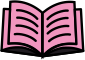For more details and the experiments with different settings for word embeddings, look at this paper summary.

Data augmentation alters your dataset in different ways to get alternative versions of the same training example. Data augmentation can increase

• the amount of data
Quality of your model depends a lot on your data. For deep learning models, having large datasets is very (very!) important.
• diversity of data
By giving different versions of training examples, you teach a model to be more robust to real-world data which can be of lower quality or simply a bit different from your training data. With augmented data, a model is less likely to overfit to specific types of training examples and will rely more on general patterns.

Data augmentation for images can be done easily: look at the examples below. The standard augmentations include flipping an image, geometrical transformations (e.g. rotation and stretching along some direction), covering parts of an image with different patches.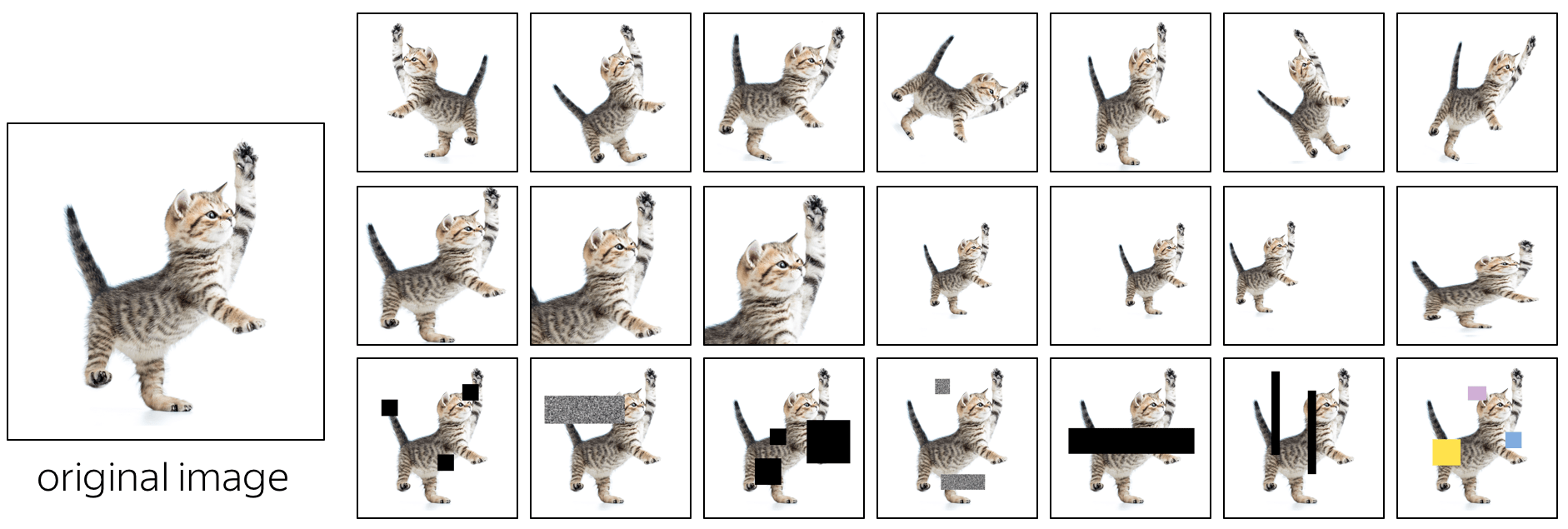### How can we do something similar for texts?

#### •word dropout - the most simple and popular

Word dropout is the simplest regularization: for each example, you choose some words randomly (say, each word is chosen with probability 10%) and replace the chosen words with either the special token UNK or with a random token from the vocabulary.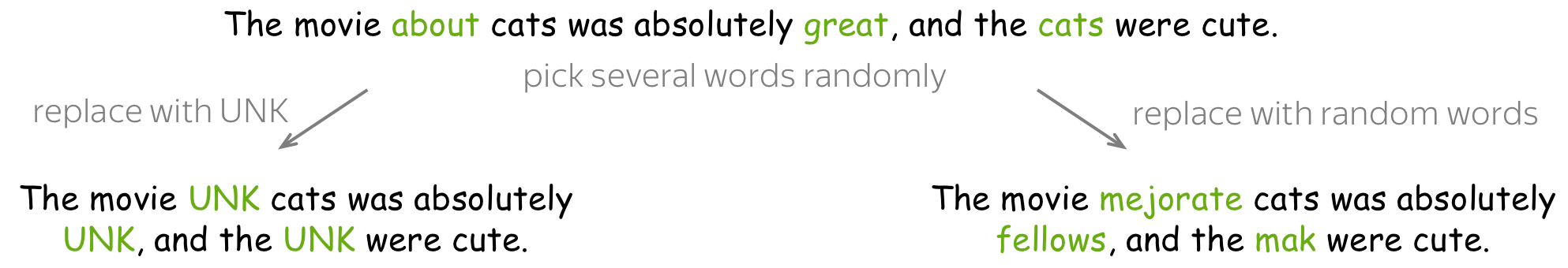The motivation here is simple: we teach a model not to over-rely on individual tokens, but take into consideration context of the whole text. For example, here we masked great, and a model has to understand the sentiment based on other words.

Note: For images, this corresponds to masking out some areas. By masking out an area of an image, we also want a model not to over-rely on local features and to make use of a more global context.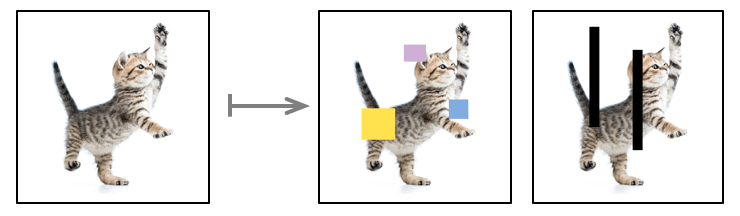#### •use external resources (e.g., thesaurus) - a bit more complicated

A bit more complicated approach is to replace words or phrases with their synonyms. The tricky part is getting these synonyms: you need external resources, and they are rarely available for languages other than English (for English, you can use e.g. WordNet). Another problem is that for languages with rich morphology (e.g., Russian) you are likely to violate the grammatical agreement.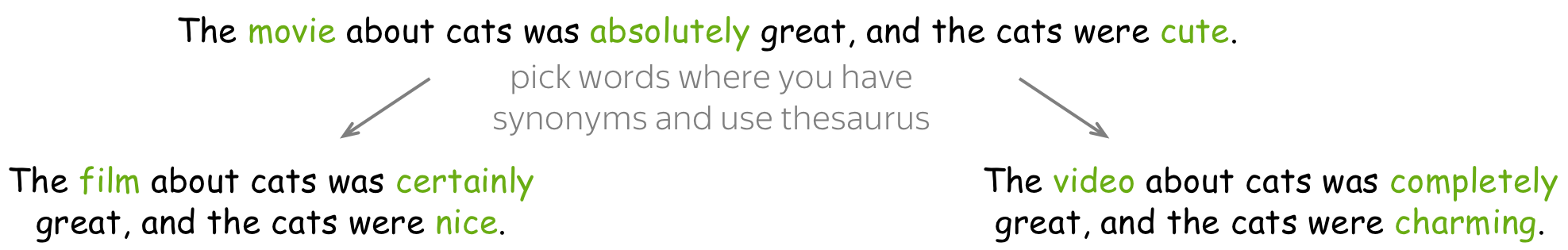#### •use separate models - even more complicated

An even more complicated method is to paraphrase the whole sentences using external models. A popular paraphrasing method is to translate a sentence to some language and back. We will learn how to train a translation model a bit later (in the Seq2seq and Attention lecture), but for now, you can use industrial systems, e.g. Yandex Translate, Google Translate, etc. (Lena: Obviously, personally I'm biased towards Yandex :) ) Note that you can combine translation systems and languages to get several paraphrases.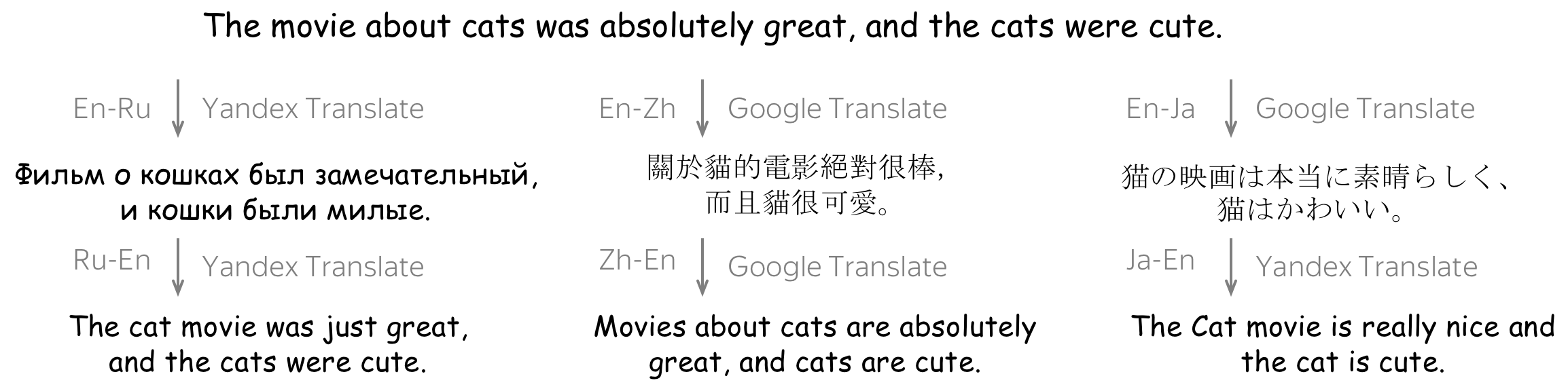Note: For images, the last two techniques correspond to geometric transformations: we want to change text, but to preserve the meaning. This is different from word dropout, where some parts are lost completely.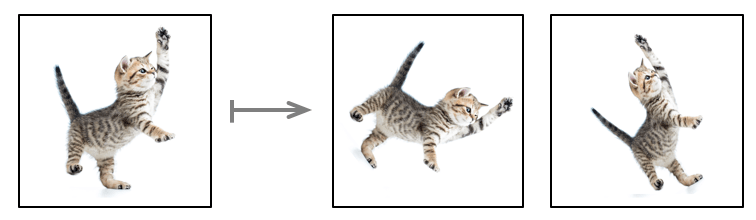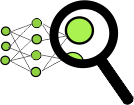# Analysis and Interpretability

## What do Convolutions Learn? Analyzing Convolutional Filters

### Convolutions in Computer Vision: Visual Patterns

Convolutions were originally developed for images, and there's already a pretty good understanding of what the filters capture and how filters from different layers from a hierarchy. While lower layers capture simple visual patterns such as lines or circles, final layers can capture the whole pictures, animals, people, etc.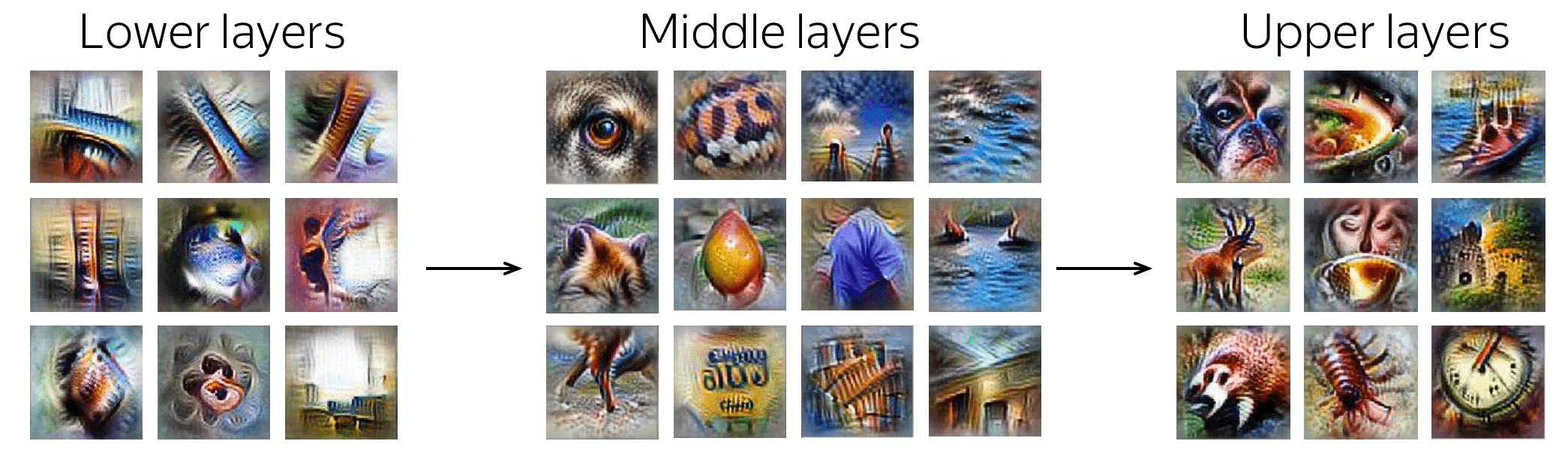Examples of patterns captured by convolution filters for images. The examples are from Activation Atlas from distill.pub.

### What About Convolutions in Texts?

This part is based on the paper Understanding Convolutional Neural Networks for Text Classification.

For images, filters capture local visual patterns which are important for classification. For text, such local patterns are word n-grams. The main findings on how CNNs work for texts are:

• convolving filters are used as ngram detectors
Each filter specializes in one or several families of closely-related ngrams. Filters are not homogeneous, i.e. a single filter can, and often does, detect multiple distinctly different families of ngrams.
• max-pooling induces a thresholding behavior
Values below a given threshold are ignored when (i.e. irrelevant to) making a prediction. For example, this paper shows that 40% of the pooled ngrams on average can be dropped with no loss of performance.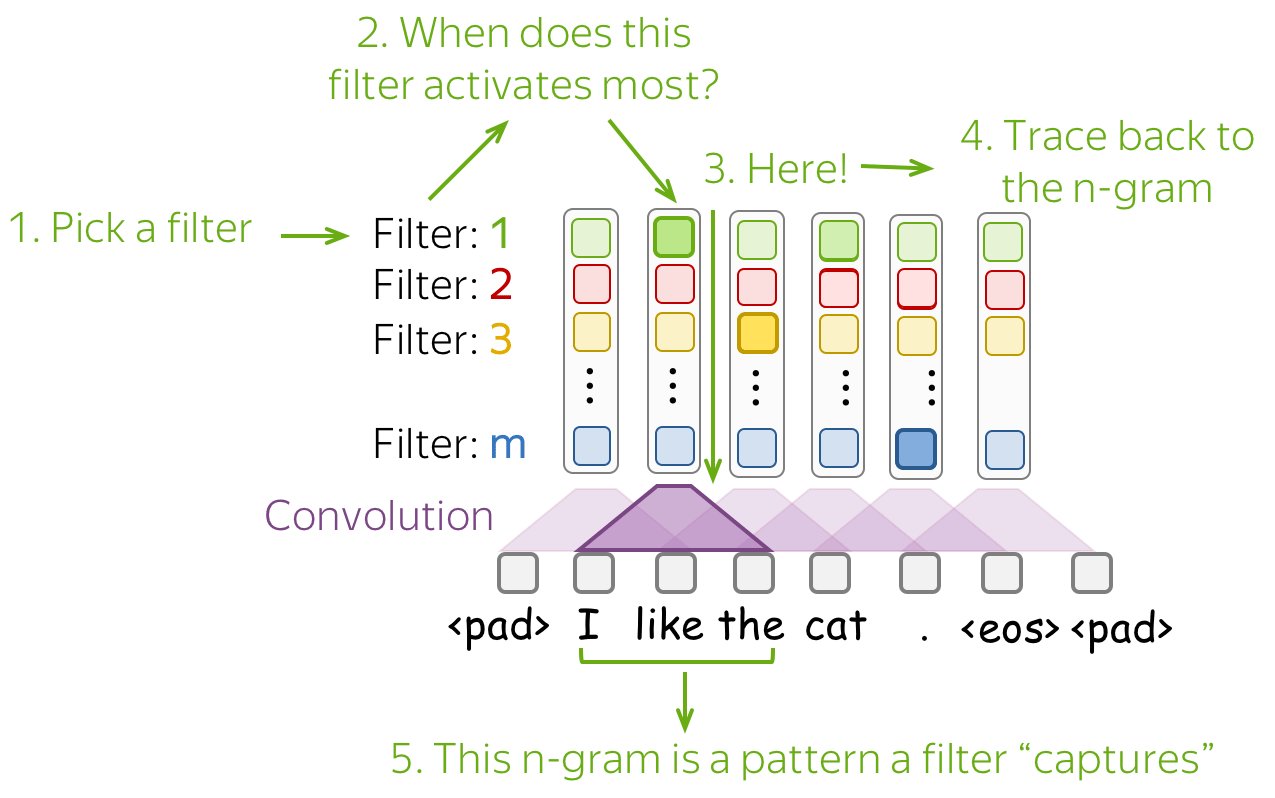The simplest way to understand what a network captures is to look which patterns activate its neurons. For convolutions, we pick a filter and find those n-grams which activate this filter most.

Below are examples of the top-1 n-gram for several filters. For one of them, we also show other n-grams which lead to high activation of this filter - you can see that the n-grams have a very similar meaning.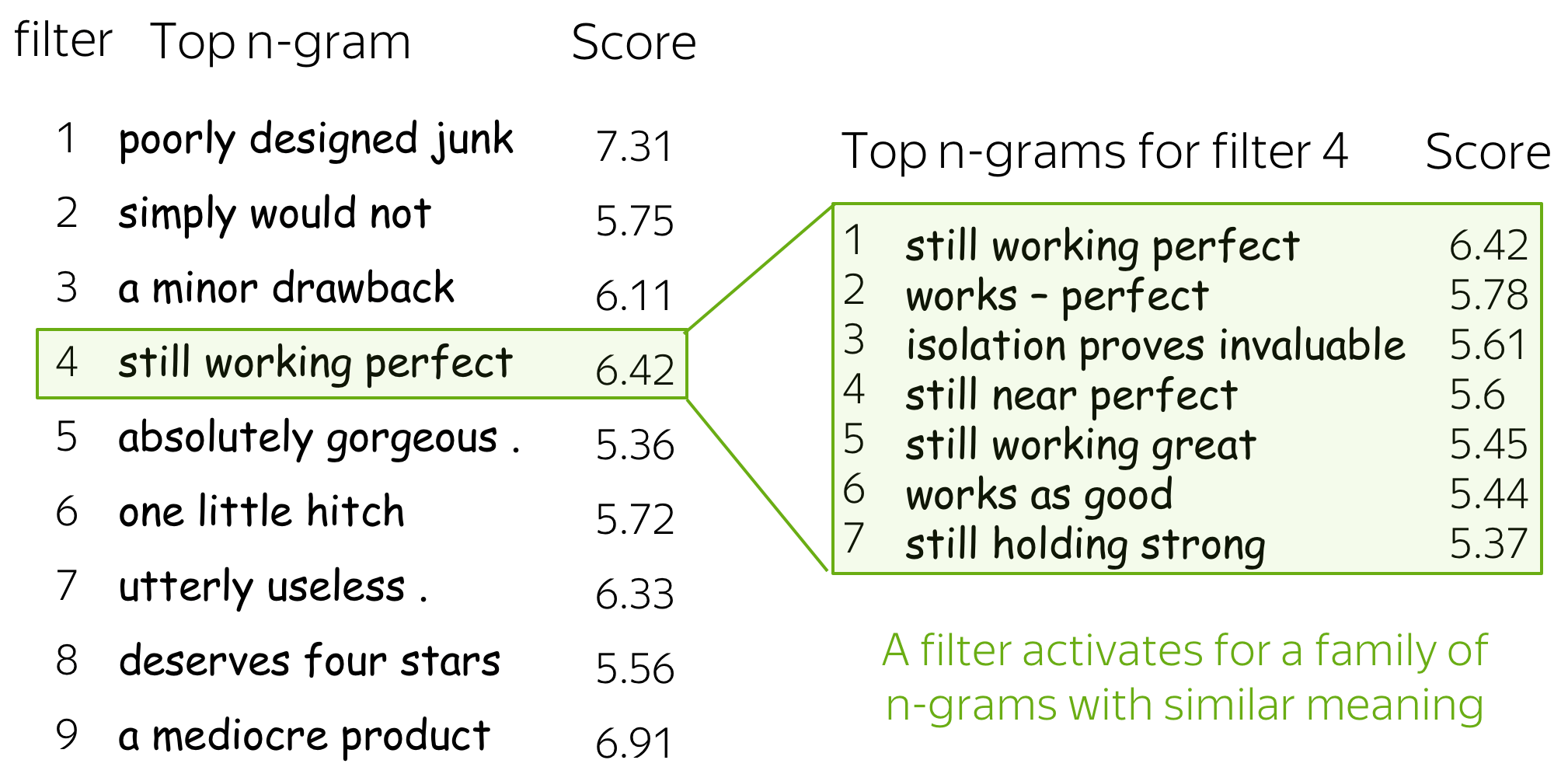For more details, look at the paper Understanding Convolutional Neural Networks for Text Classification.How RNNs trained for classification process text? Learn here.# Research Thinking

How to

• Read the short description at the beginning - this is our starting point, something known.
• Read a question and think: for a minute, a day, a week, ... - give yourself some time! Even if you are not thinking about it constantly, something can still come to mind.
• Look at the possible answers - previous attempts to answer/solve this problem.
Important: You are not supposed to come up with something exactly like here - remember, each paper usually takes the authors several months of work. It's a habit of thinking about these things that counts! All the rest a scientist needs is time: to try-fail-think until it works.

It's well-known that you will learn something easier if you are not just given the answer right away, but if you think about it first. Even if you don't want to be a researcher, this is still a good way to learn things!

## Classical Approaches

Improve Naive Bayes

The simplest Naive Bayes implementation uses tokens as features. However, this is not always good: completely different texts can have the same features.? In the example above we see the main problem of Naive Bayes: it knows nothing about context. Of course, we can not remove the "naive" assumptions (otherwise, it won't be Naive Bayes anymore). But can we improve the feature extraction part?

### Idea: Add Frequent N-Grams to the Features!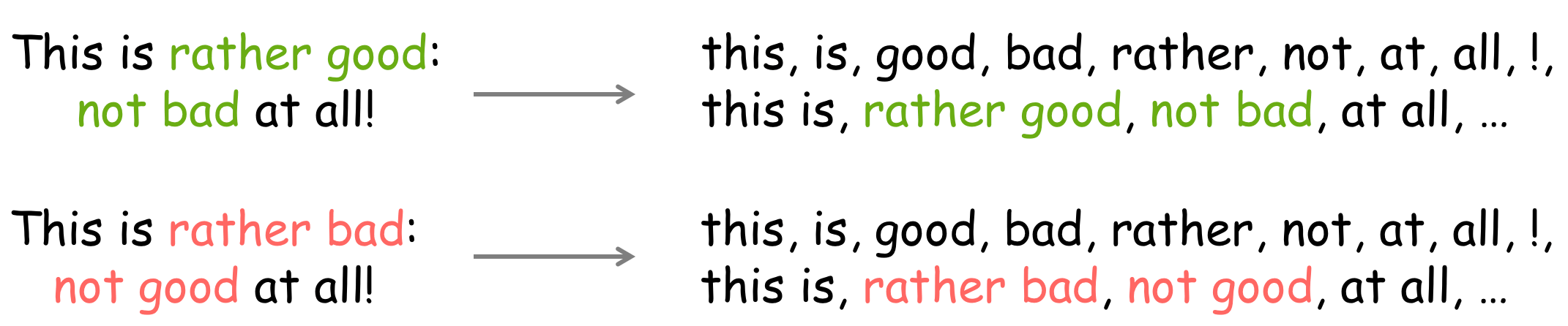Instead of using only words as features, we can also use word n-grams. Since using all n-grams would be inefficient, we can add only the frequent ones. This can fix some examples with simple negation as the one shown above.

? What other types of features you can come up with?

Note that Naive Bayes can use any categorical features - you can do anything as long as you can compute the counts for probabilities. For example,

• text length
Who knows - maybe positive reviews are longer than negative? Don't forget to categorize it, e.g., 1-20 tokens correspond to one feature, 21-30 tokens - to another, etc.
• token frequency
It may be worth checking - positive or negative reviews use more peculiar words? You can come up with some characteristics of tokens frequency: minimum of maximum, average, etc. But again - you have to categorize it!
• syntactical features (if you don't know what it is yet, skip this)
Dependency tree depth (maximum/minimum/average) - this can be a proxy of text complexity.
• anything else you can come up with
Just try :)

? Are all words equally needed for classification? If not, how can we modify the method?

### Idea: Do Not Use Unimportant Words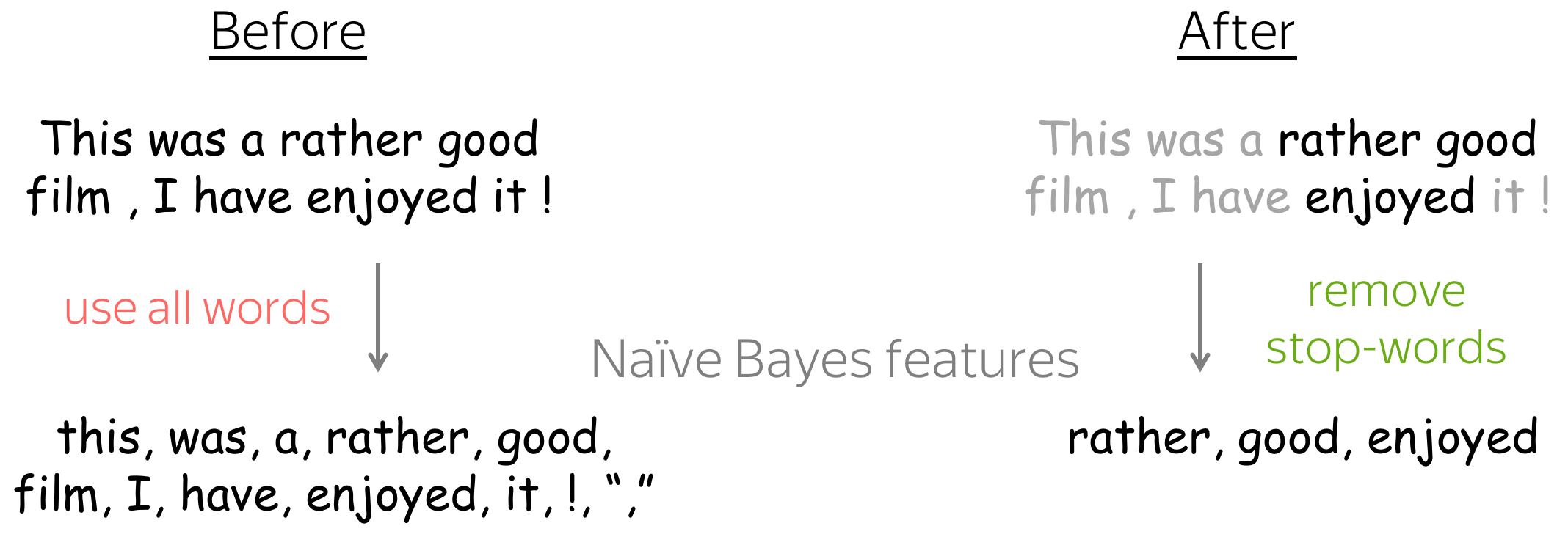If you know which words definitely do not influence class probability, you can remove them from the features! For example, we can remove stop-words: determiners, prepositions, etc.

Note: you need to be really careful - don't remove something useful!

## Neural Approaches

Fine-tuning embeddings: Why and when can this help?

Before training models, you can first think why fine-tuning can be useful, and which types of examples can benefit from it. Remember how embeddings are trained: words that are used similarly in texts have very close embeddings. Therefore, sometimes antonyms are closest to each other, e.g. descent and ascent.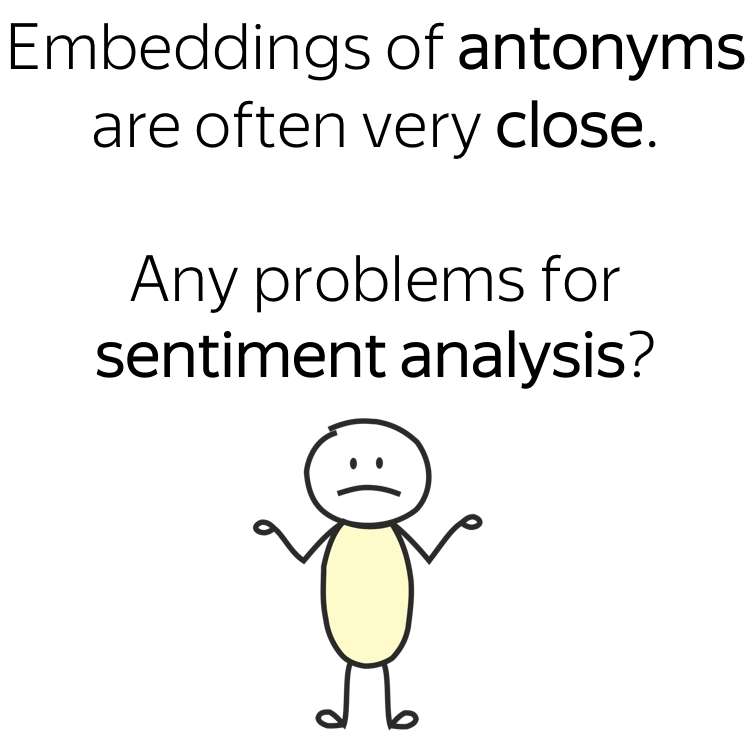? Imagine we want to use embeddings for sentiment classification. Can you find examples of antonyms such that if their embeddings are very close, it would hurt sentiment classification? If you can, it means that might be better to fine-tune!

### Without fine-tuning, closest to bad is good!

The figure shows closest neighbors of Word2Vec embeddings before and after fine-tuning (examples are taken from this paper).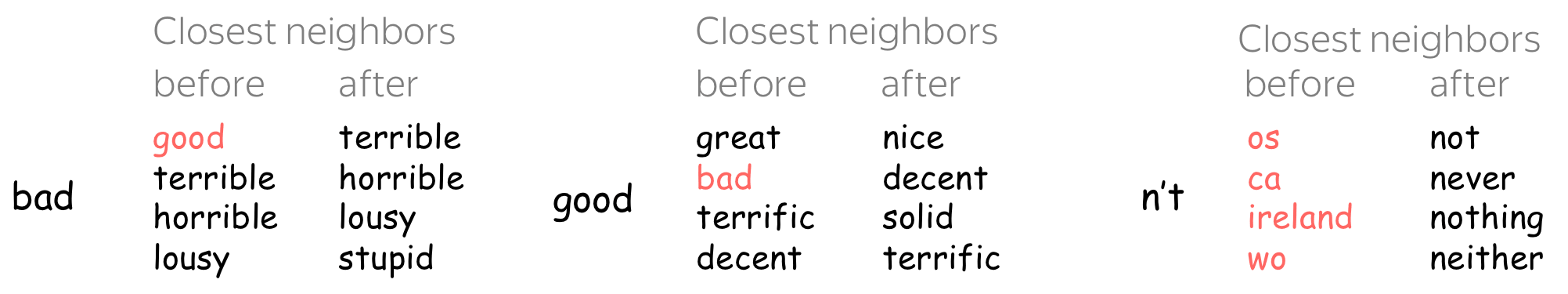Without fine-tuning, closest to bad is good! Without fine-tuning, it would be very hard for a model to separate positive and negative using these embeddings. This is only one example of antonyms with close embeddings which can hurt sentiment classification.

Fine-tuning can also help to improve understanding of tokens such as n't: rare in the corpus word embeddings were trained on, but not rare in the corpus we care about. More generally, if your task-specific domain is different from the word embeddings training data, fine-tuning is a good idea.

Here will be more exercises!

This part will be expanding from time to time.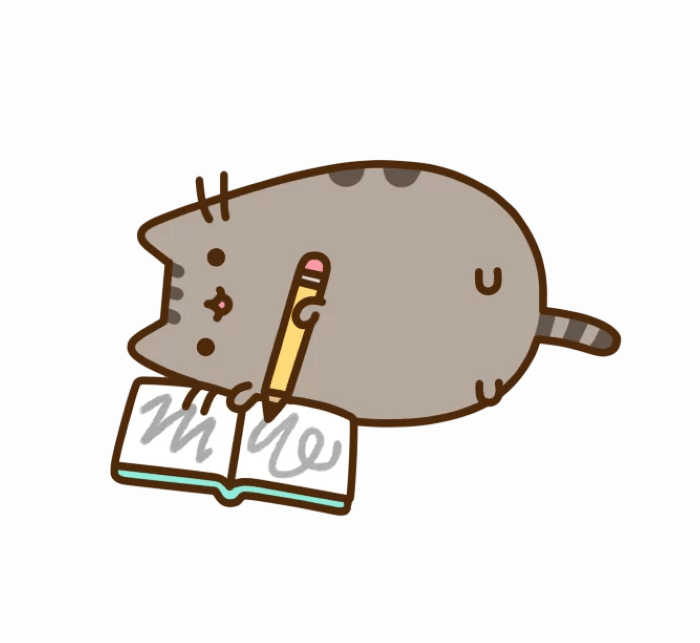# Have Fun!

Coming soon!

We are still working on this!